Скачать презентацию Modern Atomic Theory a k a the electron

78113368a2887c2d6c3f03d49746402e.ppt

• Количество слайдов: 89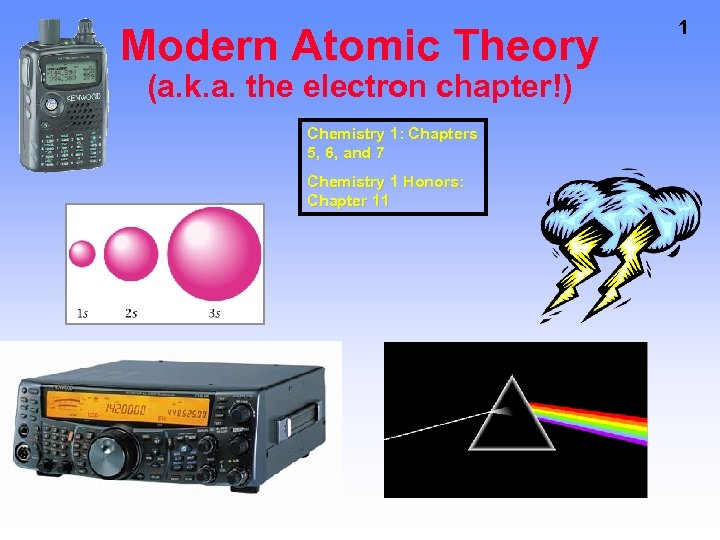Modern Atomic Theory (a. k. a. the electron chapter!) Chemistry 1: Chapters 5, 6, and 7 Chemistry 1 Honors: Chapter 11 1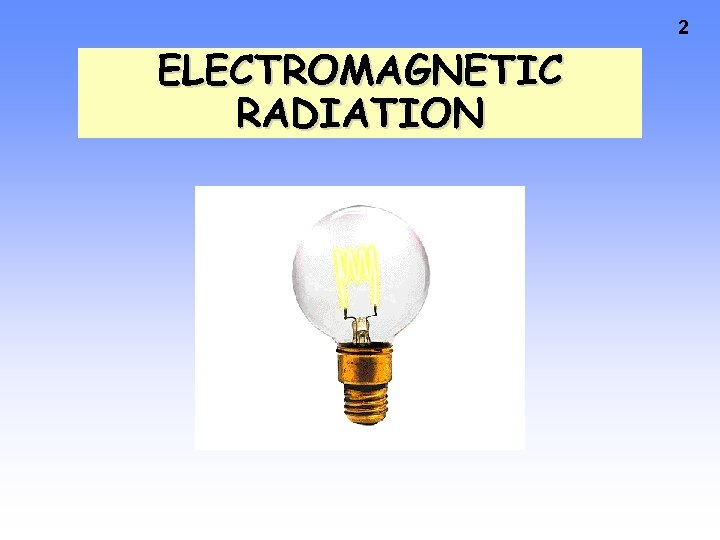2 ELECTROMAGNETIC RADIATION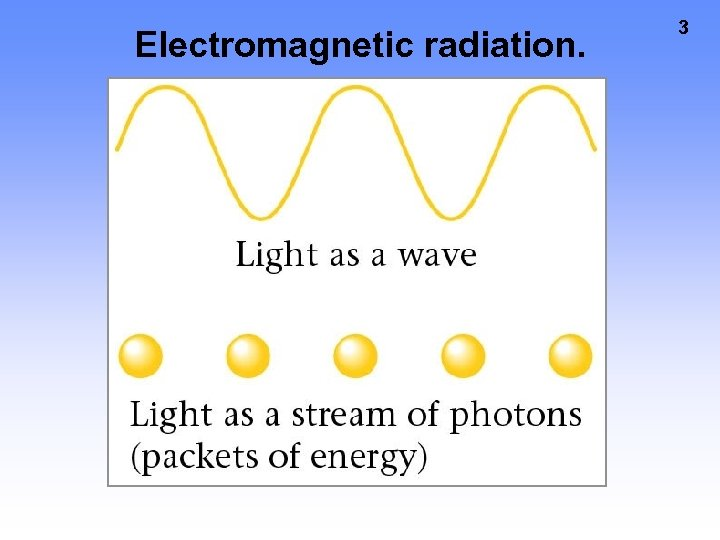Electromagnetic radiation. 3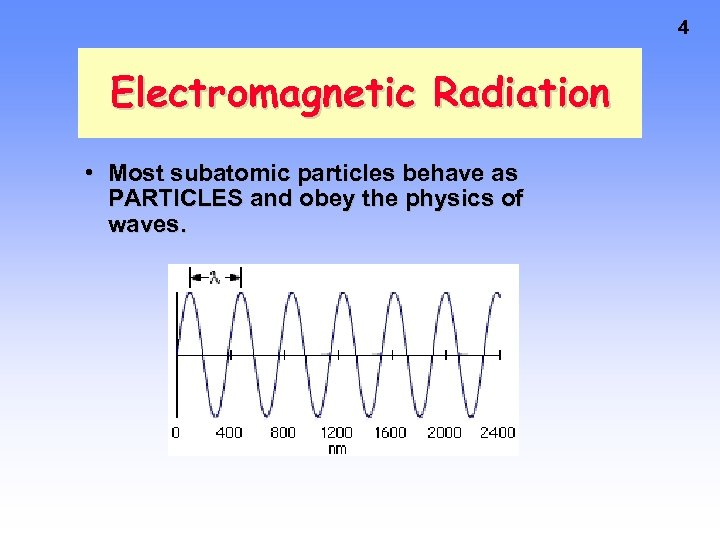4 Electromagnetic Radiation • Most subatomic particles behave as PARTICLES and obey the physics of waves.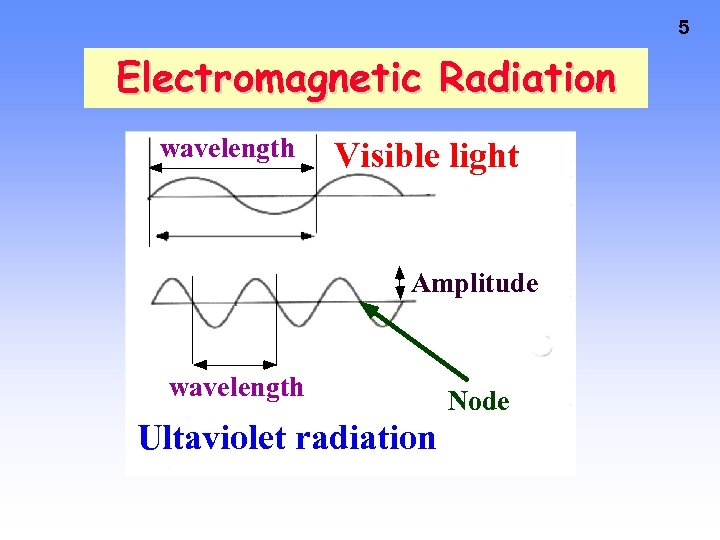5 Electromagnetic Radiation wavelength Visible light Amplitude wavelength Ultaviolet radiation Node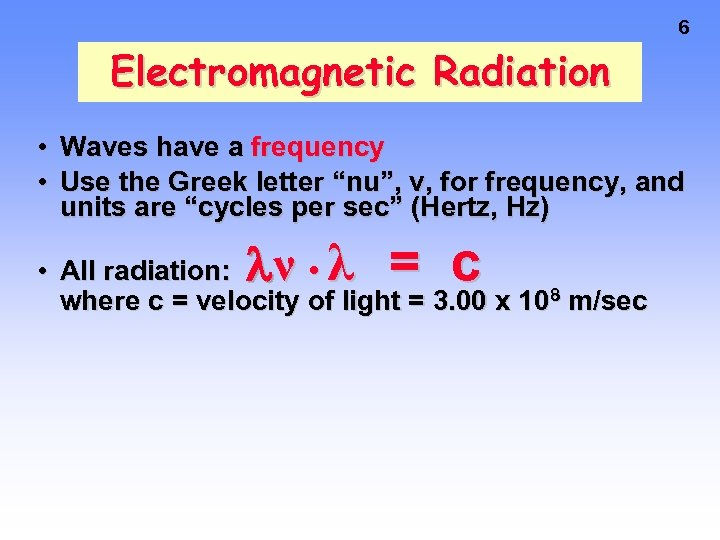6 Electromagnetic Radiation • • Waves have a frequency Use the Greek letter “nu”, ν, for frequency, and units are “cycles per sec” (Hertz, Hz) ν λ = c • All radiation: • where c = velocity of light = 3. 00 x 108 m/sec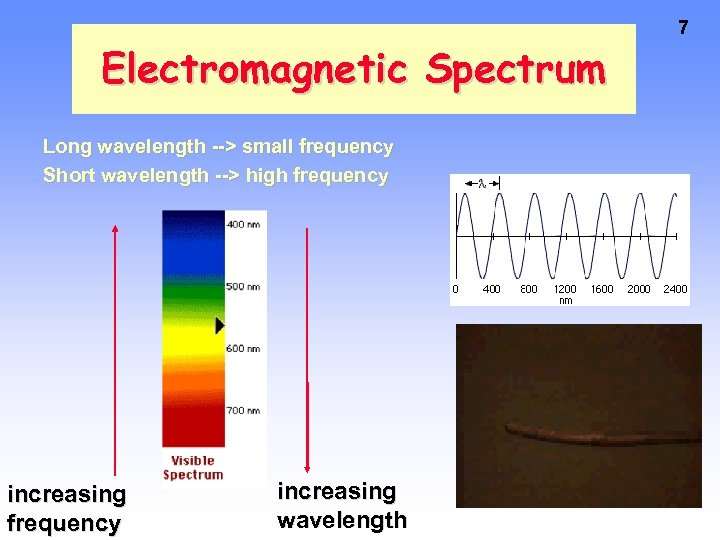Electromagnetic Spectrum Long wavelength --> small frequency Short wavelength --> high frequency increasing wavelength 7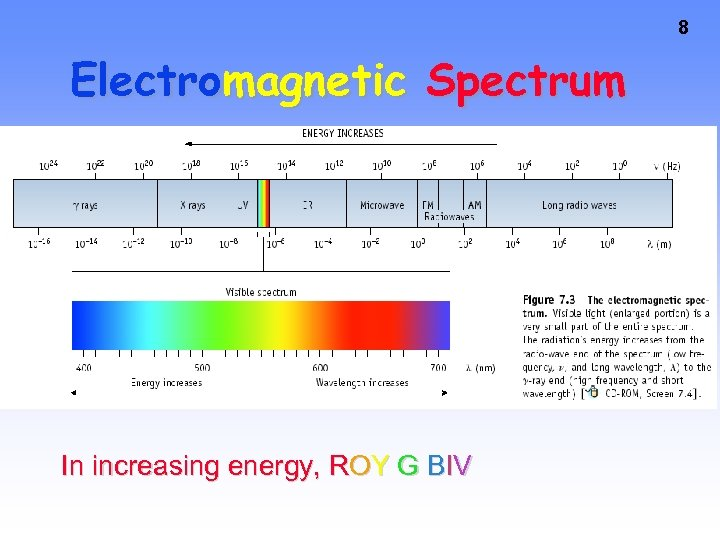8 Electromagnetic Spectrum In increasing energy, ROY G BIV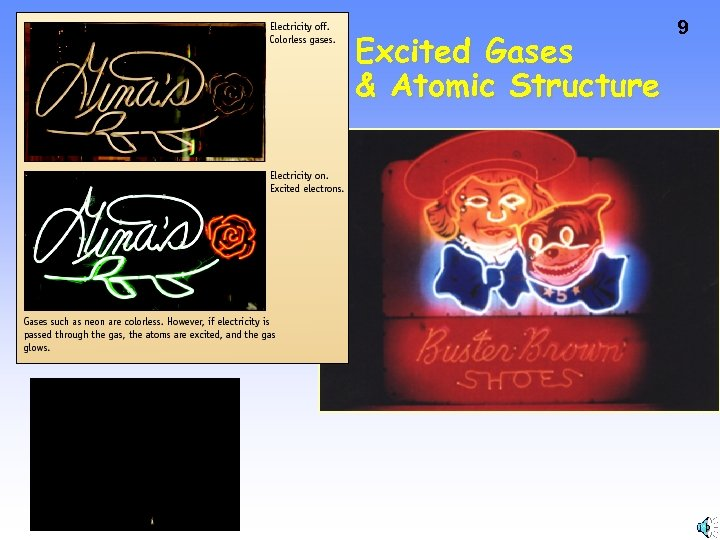Excited Gases & Atomic Structure 9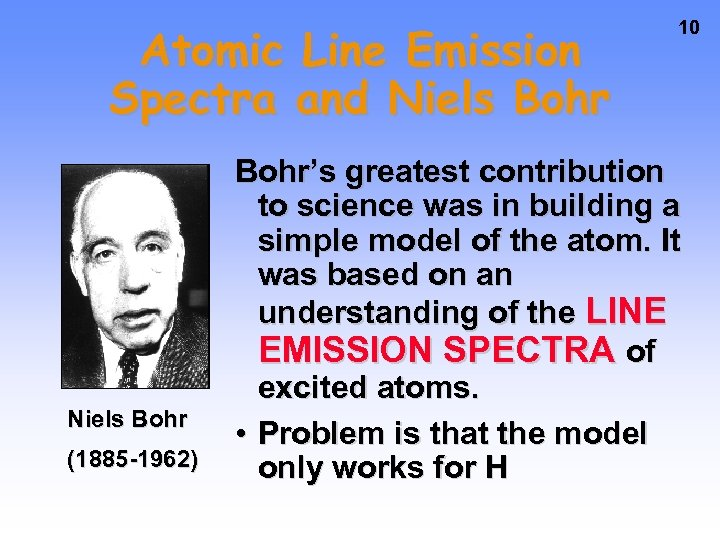Atomic Line Emission Spectra and Niels Bohr (1885 -1962) 10 Bohr’s greatest contribution to science was in building a simple model of the atom. It was based on an understanding of the LINE EMISSION SPECTRA of excited atoms. • Problem is that the model only works for H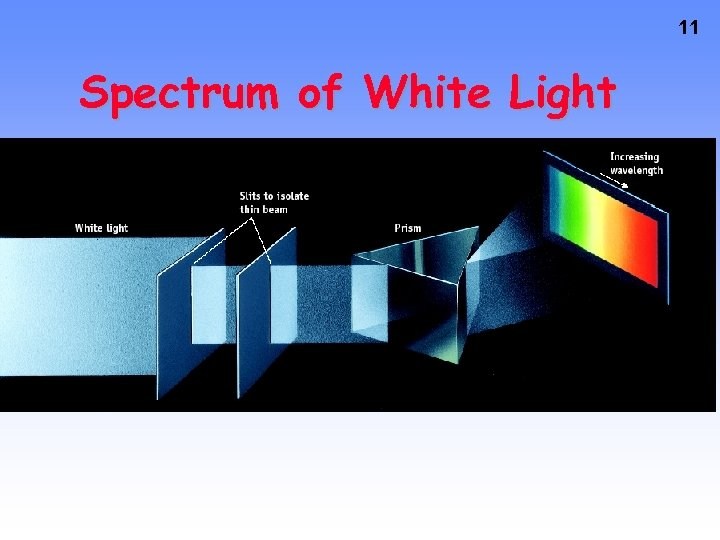11 Spectrum of White Light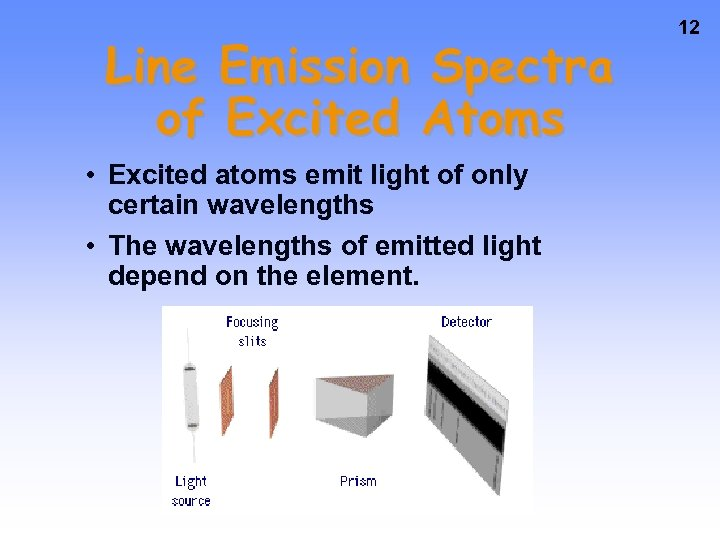Line Emission Spectra of Excited Atoms • Excited atoms emit light of only certain wavelengths • The wavelengths of emitted light depend on the element. 12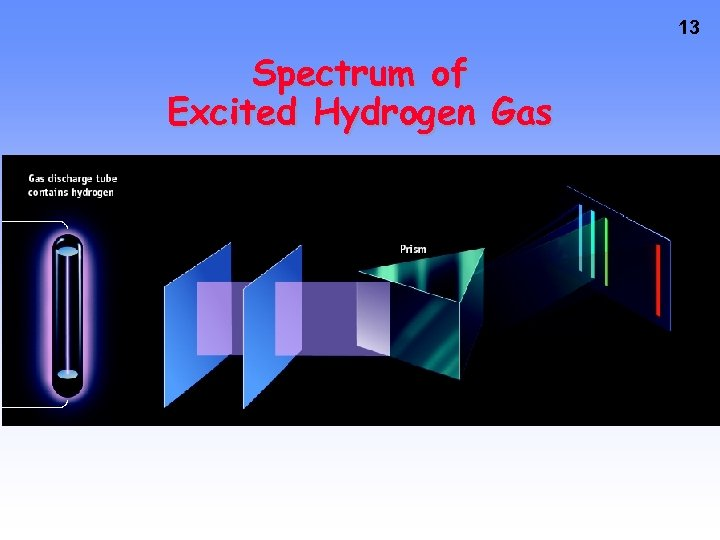13 Spectrum of Excited Hydrogen Gas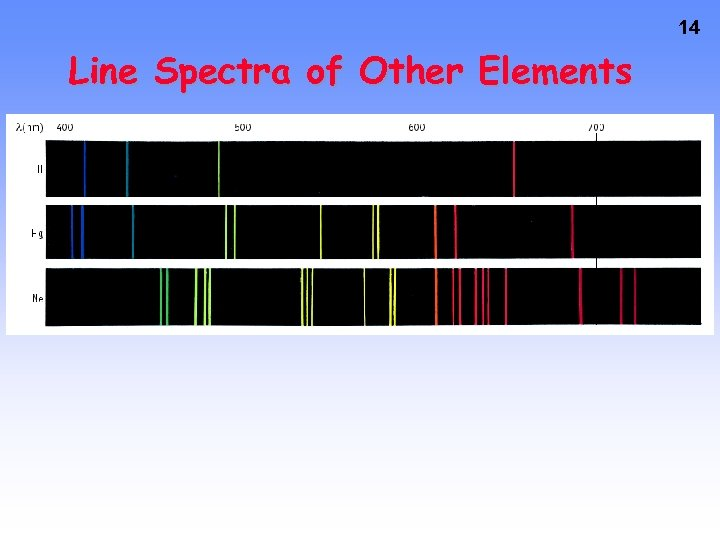14 Line Spectra of Other Elements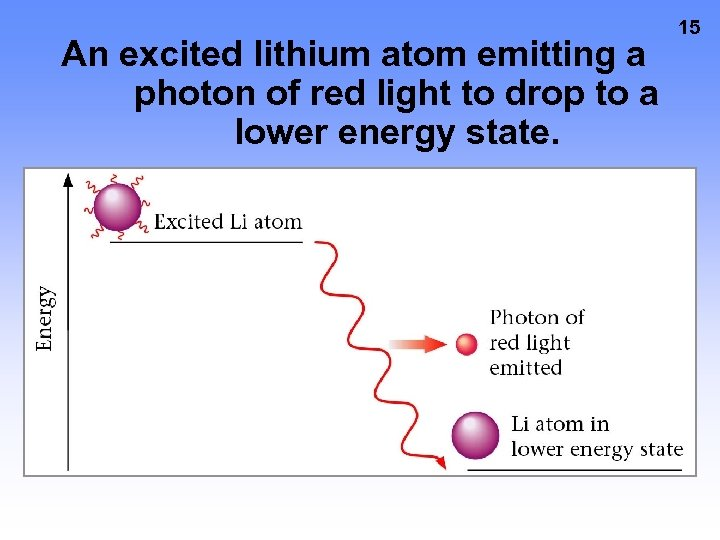An excited lithium atom emitting a photon of red light to drop to a lower energy state. 15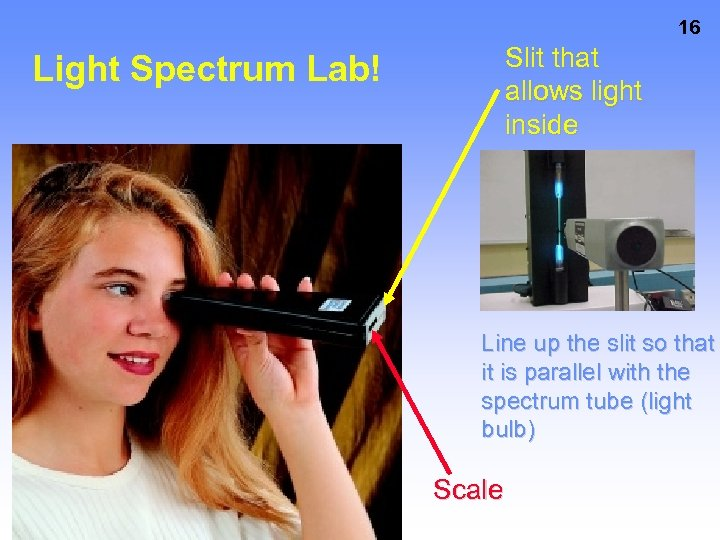16 Slit that allows light inside Light Spectrum Lab! Line up the slit so that it is parallel with the spectrum tube (light bulb) Scale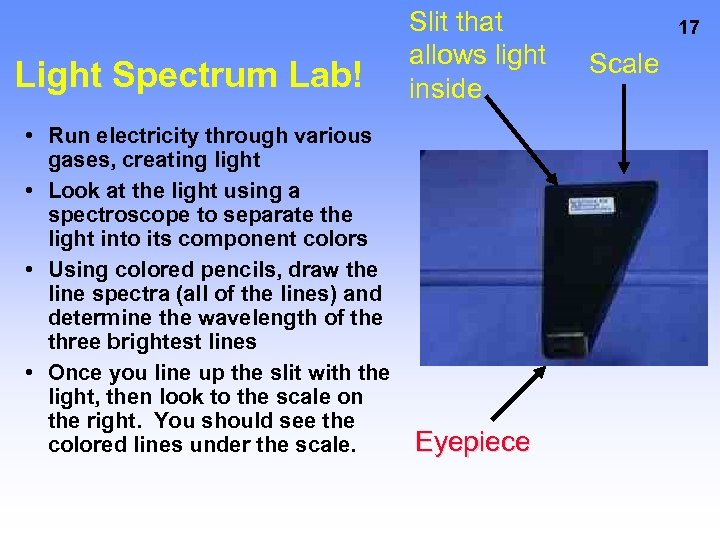Light Spectrum Lab! • Run electricity through various gases, creating light • Look at the light using a spectroscope to separate the light into its component colors • Using colored pencils, draw the line spectra (all of the lines) and determine the wavelength of the three brightest lines • Once you line up the slit with the light, then look to the scale on the right. You should see the colored lines under the scale. Slit that allows light inside Eyepiece 17 Scale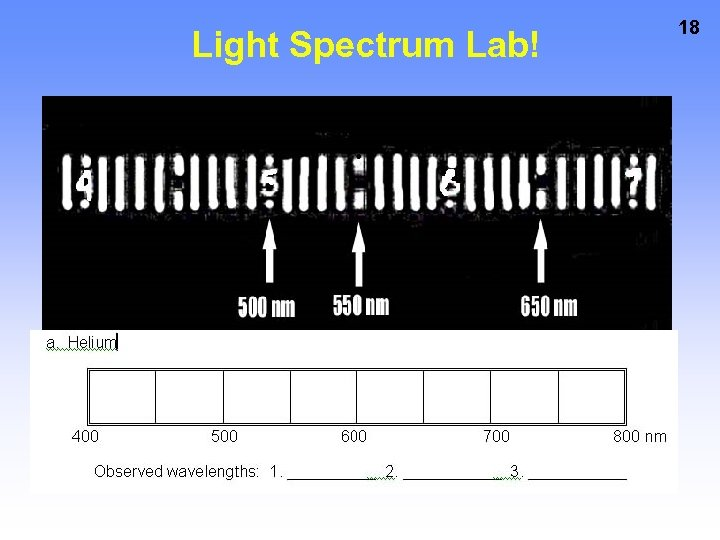Light Spectrum Lab! 18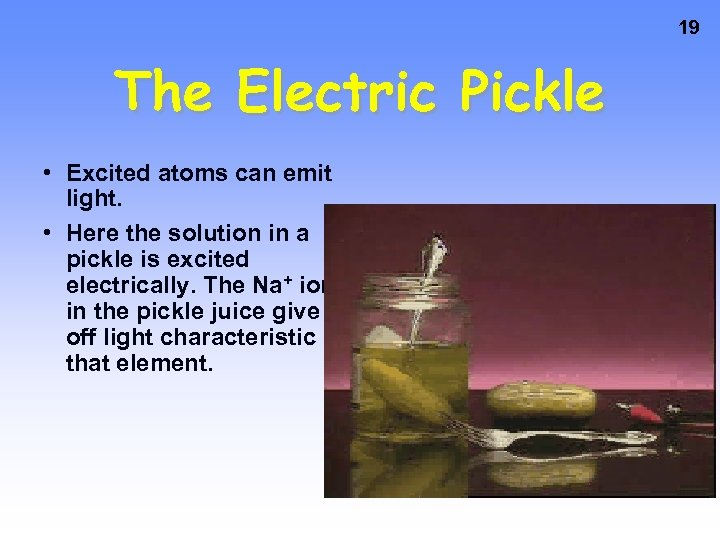19 The Electric Pickle • Excited atoms can emit light. • Here the solution in a pickle is excited electrically. The Na+ ions in the pickle juice give off light characteristic of that element.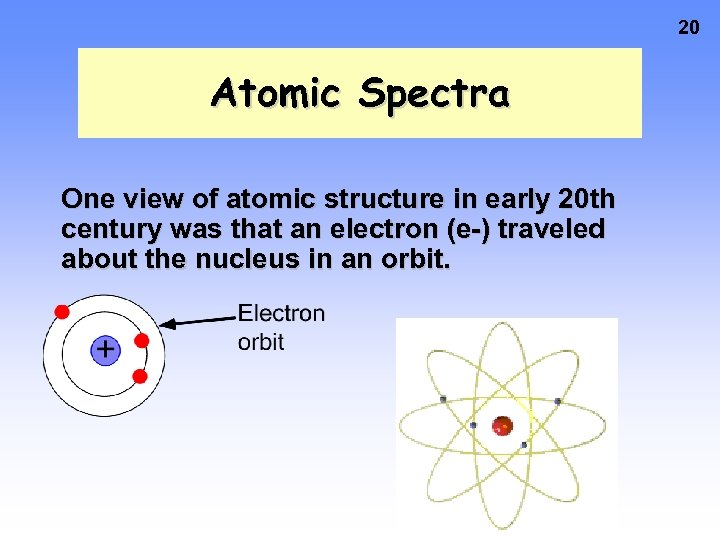20 Atomic Spectra One view of atomic structure in early 20 th century was that an electron (e-) traveled about the nucleus in an orbit.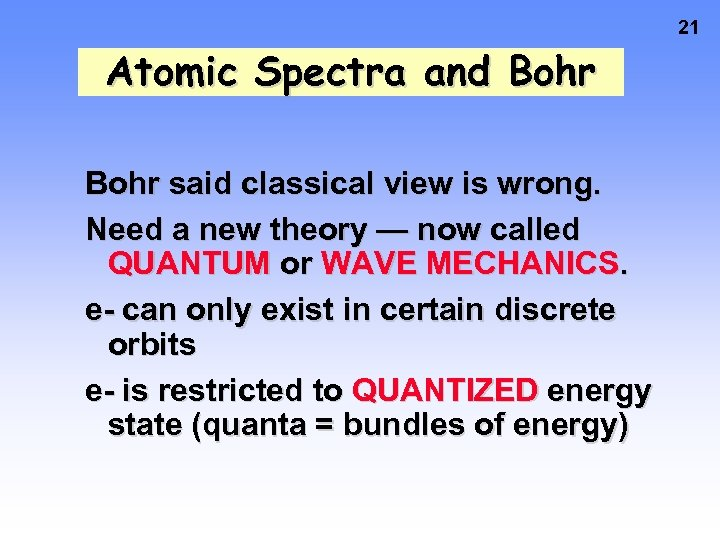21 Atomic Spectra and Bohr said classical view is wrong. Need a new theory — now called QUANTUM or WAVE MECHANICS. e- can only exist in certain discrete orbits e- is restricted to QUANTIZED energy state (quanta = bundles of energy)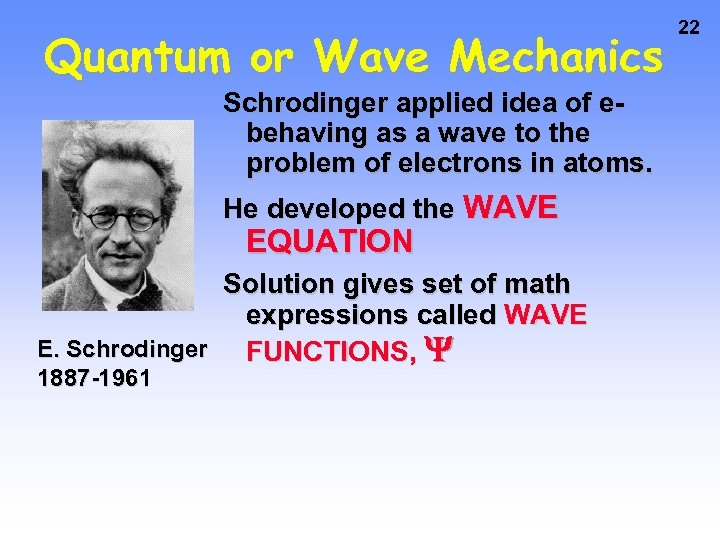Quantum or Wave Mechanics Schrodinger applied idea of ebehaving as a wave to the problem of electrons in atoms. He developed the WAVE EQUATION Solution gives set of math expressions called WAVE E. Schrodinger FUNCTIONS, 1887 -1961 22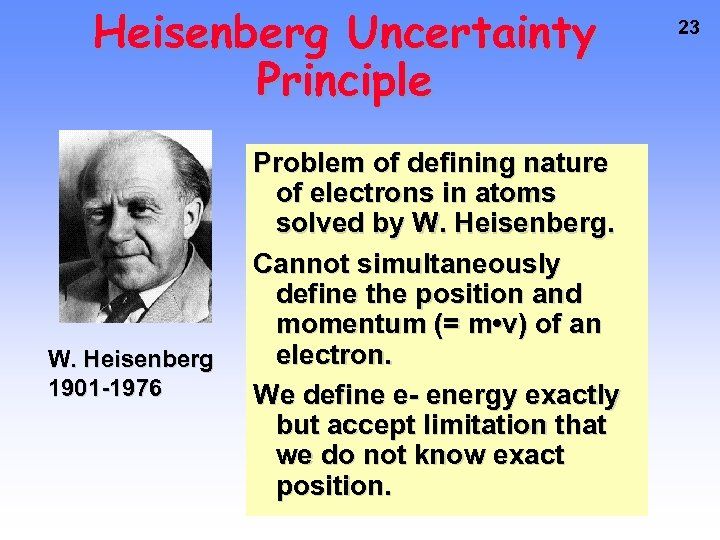Heisenberg Uncertainty Principle W. Heisenberg 1901 -1976 Problem of defining nature of electrons in atoms solved by W. Heisenberg. Cannot simultaneously define the position and momentum (= m • v) of an electron. We define e- energy exactly but accept limitation that we do not know exact position. 23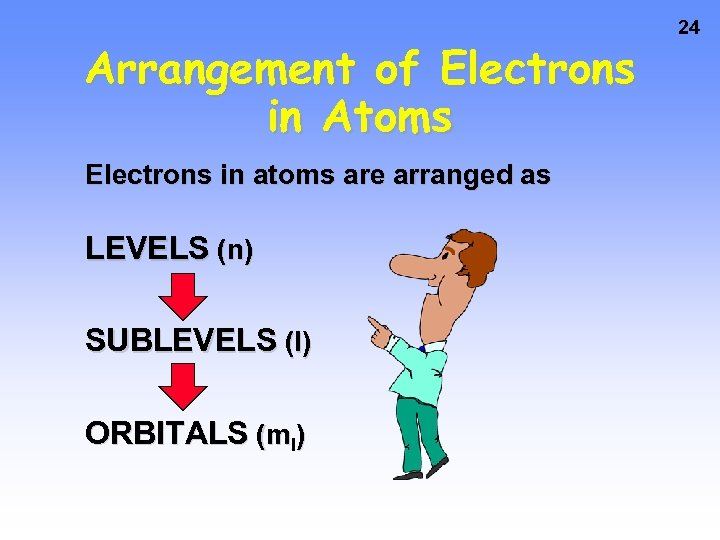Arrangement of Electrons in Atoms Electrons in atoms are arranged as LEVELS (n) SUBLEVELS (l) ORBITALS (ml) 24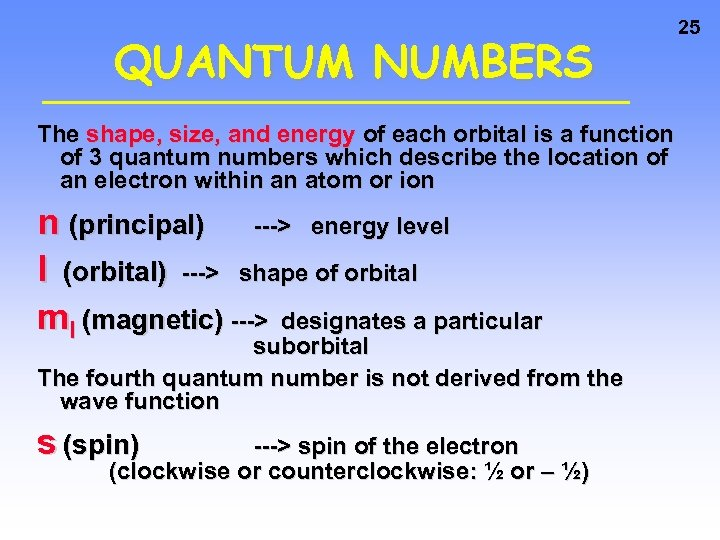QUANTUM NUMBERS The shape, size, and energy of each orbital is a function of 3 quantum numbers which describe the location of an electron within an atom or ion n (principal) ---> energy level l (orbital) ---> shape of orbital ml (magnetic) ---> designates a particular suborbital The fourth quantum number is not derived from the wave function s (spin) ---> spin of the electron (clockwise or counterclockwise: ½ or – ½) 25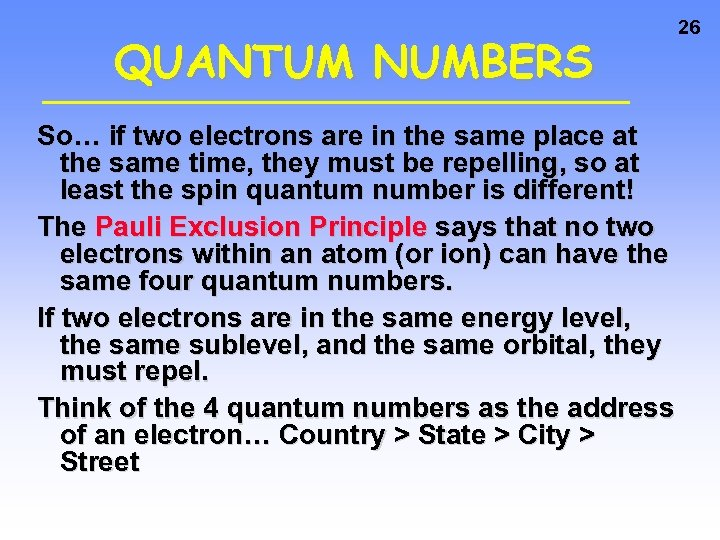QUANTUM NUMBERS So… if two electrons are in the same place at the same time, they must be repelling, so at least the spin quantum number is different! The Pauli Exclusion Principle says that no two electrons within an atom (or ion) can have the same four quantum numbers. If two electrons are in the same energy level, the same sublevel, and the same orbital, they must repel. Think of the 4 quantum numbers as the address of an electron… Country > State > City > Street 26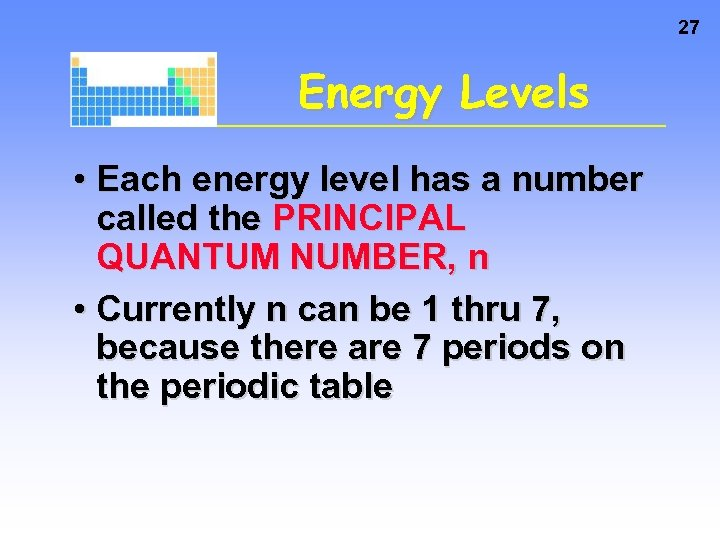27 Energy Levels • Each energy level has a number called the PRINCIPAL QUANTUM NUMBER, n • Currently n can be 1 thru 7, because there are 7 periods on the periodic table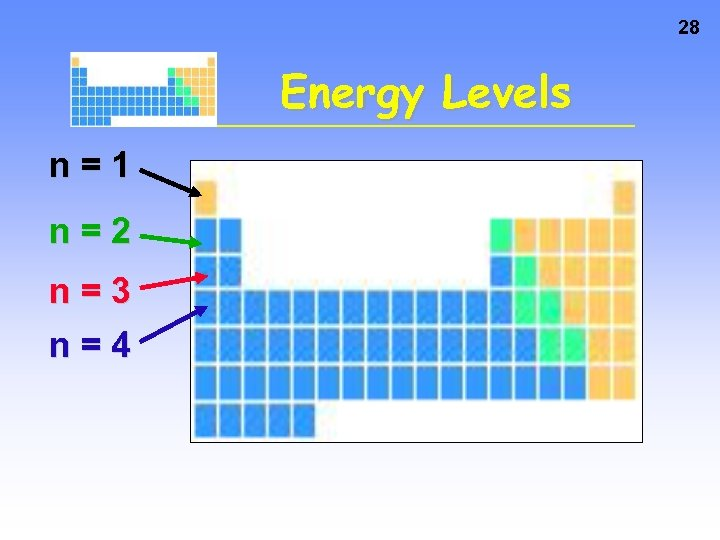28 Energy Levels n=1 n=2 n=3 n=4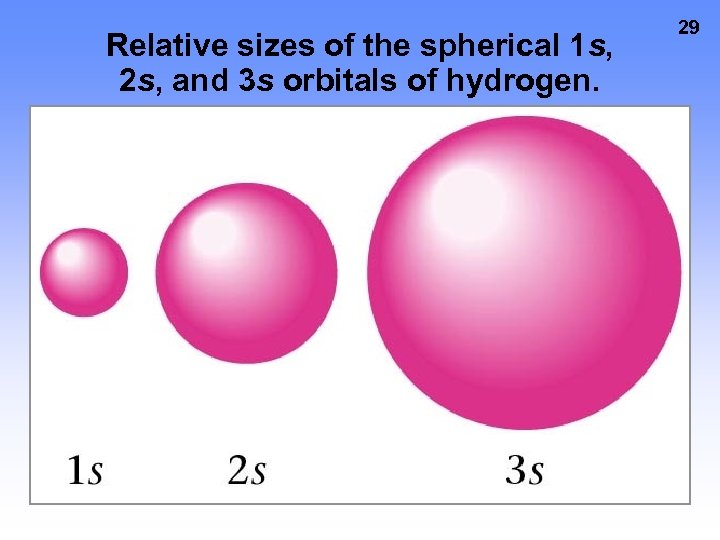Relative sizes of the spherical 1 s, 2 s, and 3 s orbitals of hydrogen. 29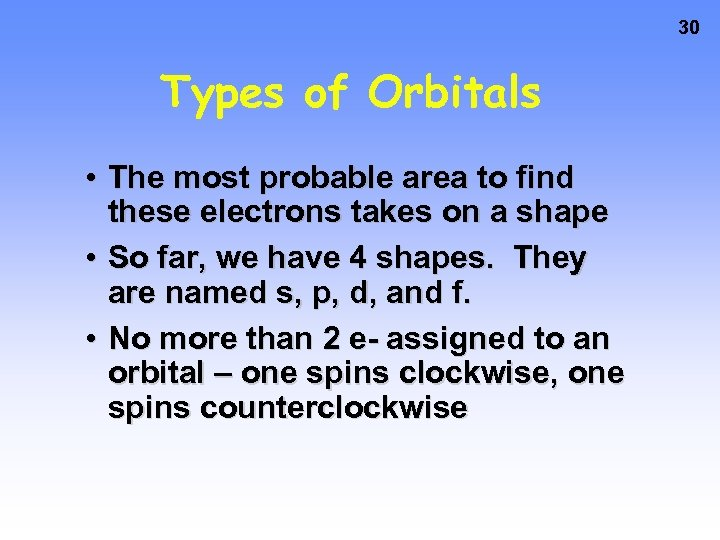30 Types of Orbitals • The most probable area to find these electrons takes on a shape • So far, we have 4 shapes. They are named s, p, d, and f. • No more than 2 e- assigned to an orbital – one spins clockwise, one spins counterclockwise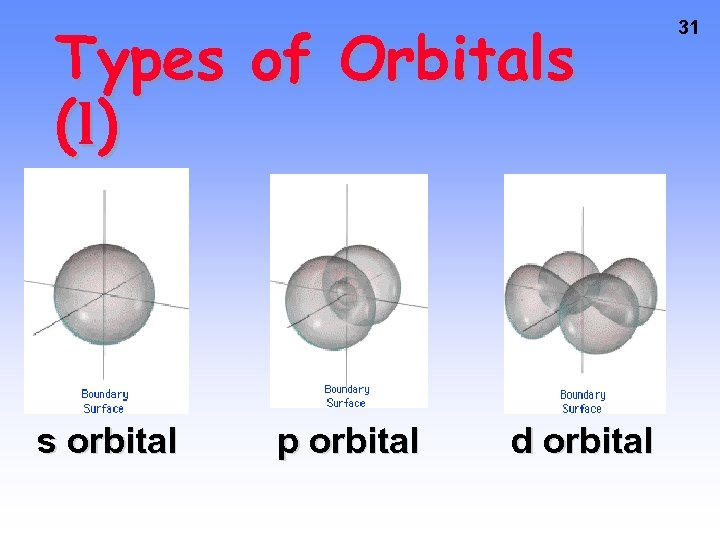Types of Orbitals ( l) s orbital p orbital d orbital 31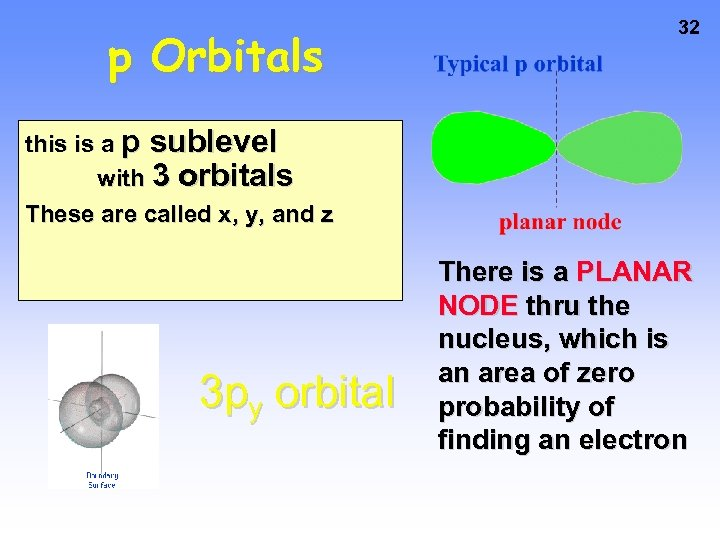p Orbitals 32 this is a p sublevel with 3 orbitals These are called x, y, and z 3 py orbital There is a PLANAR NODE thru the nucleus, which is an area of zero probability of finding an electron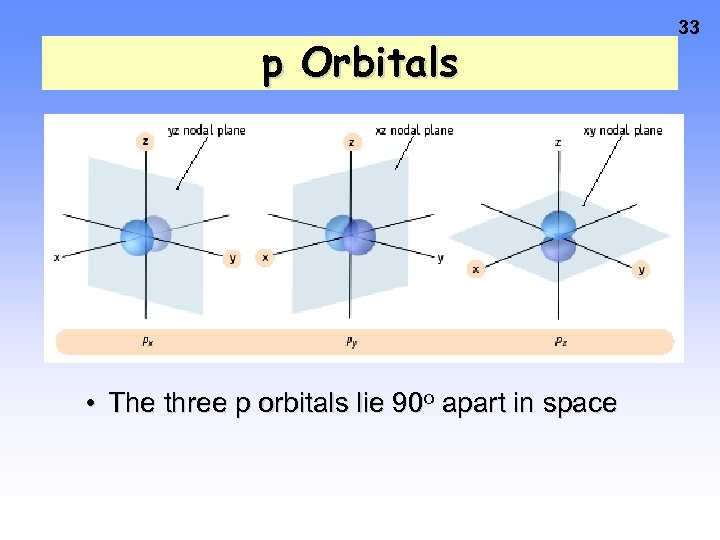p Orbitals • The three p orbitals lie 90 o apart in space 33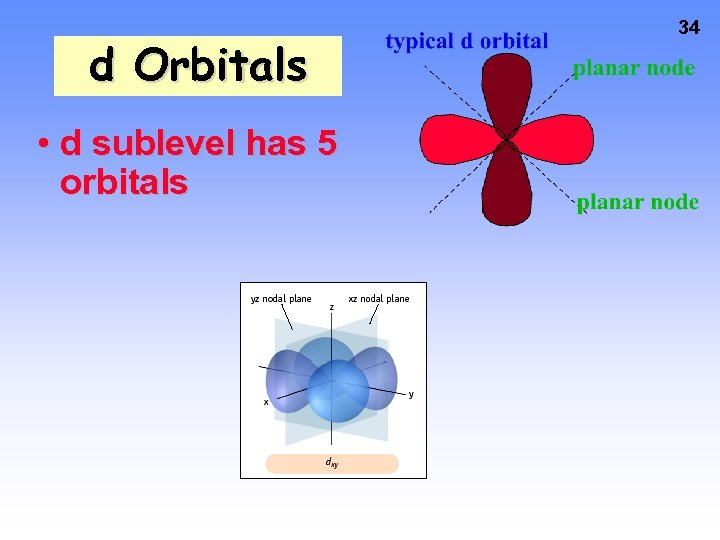d Orbitals • d sublevel has 5 orbitals 34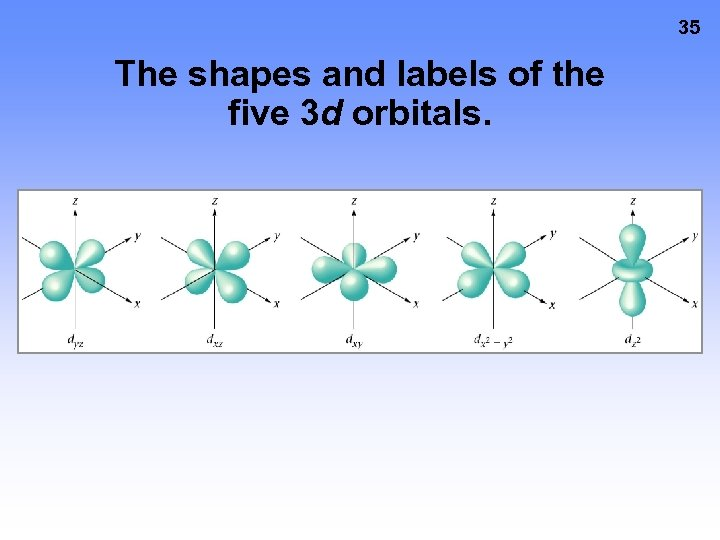35 The shapes and labels of the five 3 d orbitals.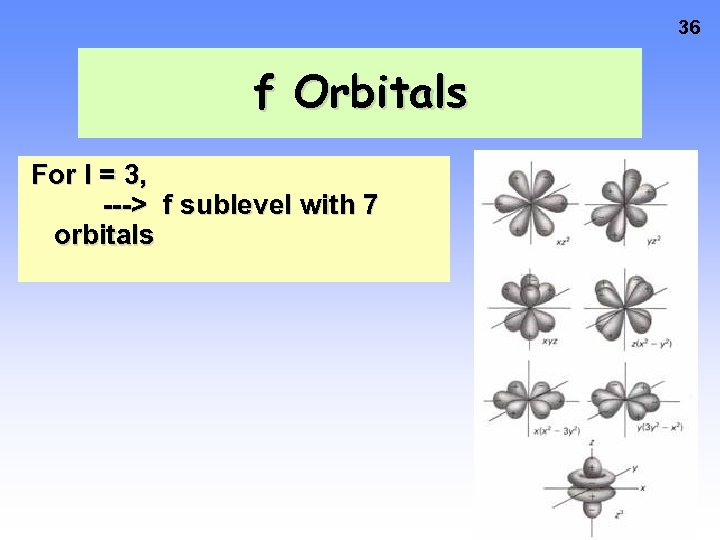36 f Orbitals For l = 3, ---> f sublevel with 7 orbitals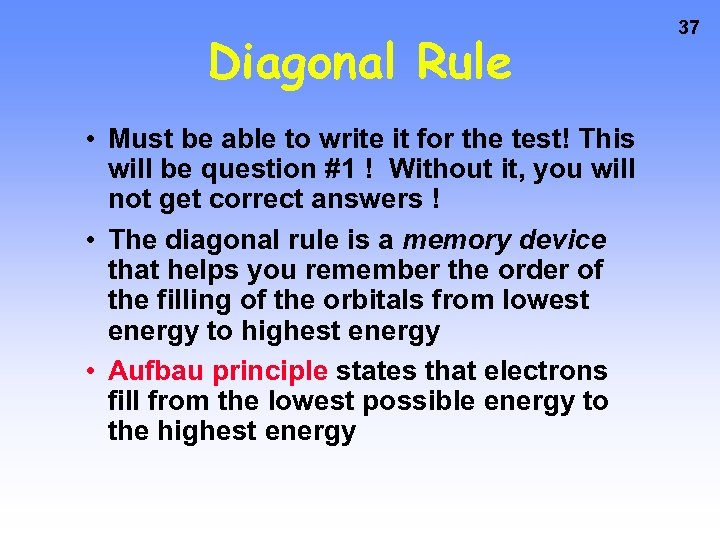Diagonal Rule • Must be able to write it for the test! This will be question #1 ! Without it, you will not get correct answers ! • The diagonal rule is a memory device that helps you remember the order of the filling of the orbitals from lowest energy to highest energy • Aufbau principle states that electrons fill from the lowest possible energy to the highest energy 37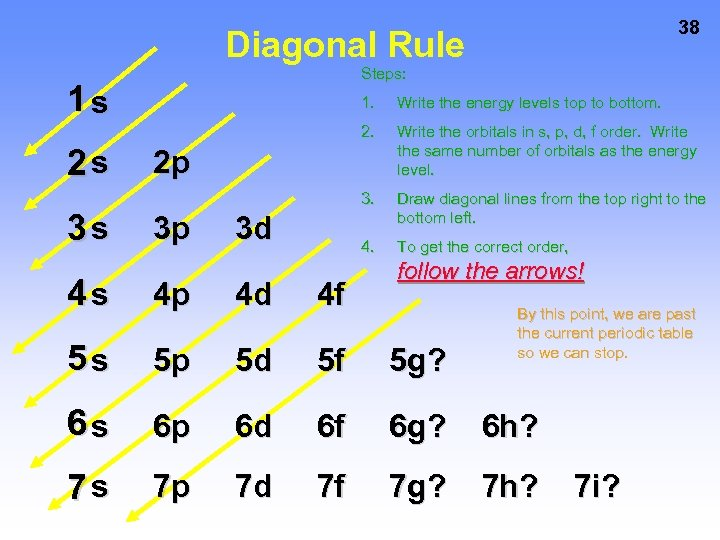38 Diagonal Rule Steps: 1 s 3 s Write the energy levels top to bottom. 2 s 1. Write the orbitals in s, p, d, f order. Write the same number of orbitals as the energy level. 3. Draw diagonal lines from the top right to the bottom left. 4. To get the correct order, 2 p 3 p 3 d follow the arrows! 4 s 4 p 4 d 4 f 5 s 5 p 5 d 5 f 5 g? 6 s 6 p 6 d 6 f 6 g? 6 h? 7 s 7 p 7 d 7 f 7 g? 7 h? By this point, we are past the current periodic table so we can stop. 7 i?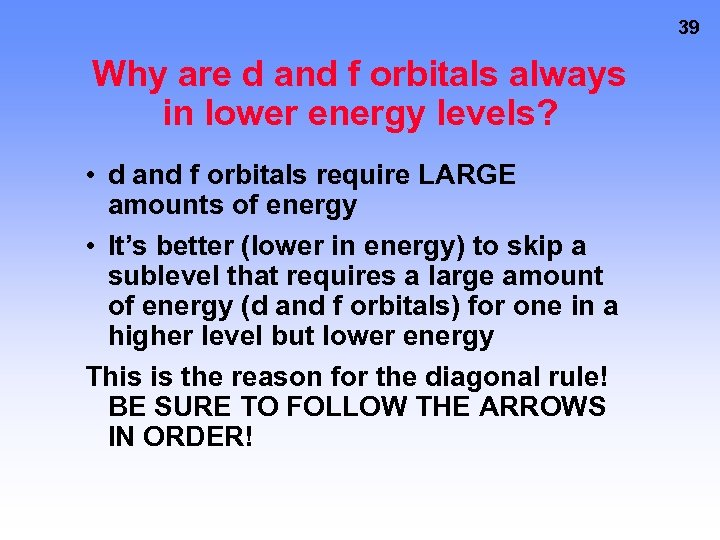39 Why are d and f orbitals always in lower energy levels? • d and f orbitals require LARGE amounts of energy • It’s better (lower in energy) to skip a sublevel that requires a large amount of energy (d and f orbitals) for one in a higher level but lower energy This is the reason for the diagonal rule! BE SURE TO FOLLOW THE ARROWS IN ORDER!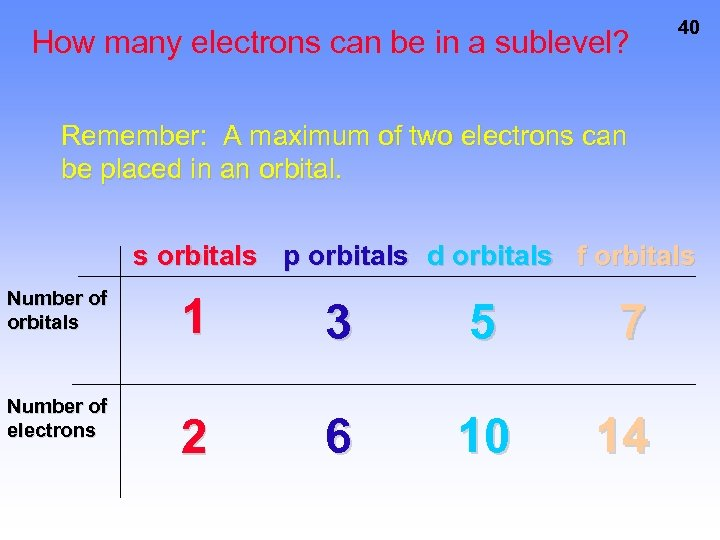How many electrons can be in a sublevel? 40 Remember: A maximum of two electrons can be placed in an orbital. s orbitals p orbitals d orbitals f orbitals Number of electrons 1 3 5 7 2 6 10 14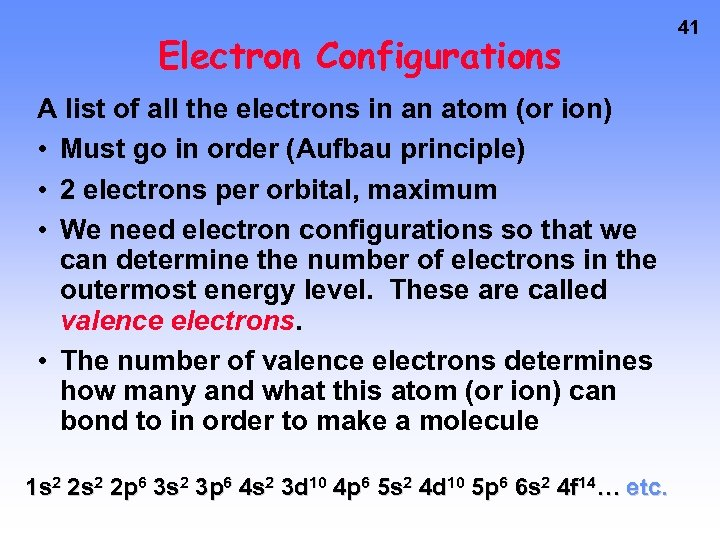Electron Configurations A list of all the electrons in an atom (or ion) • Must go in order (Aufbau principle) • 2 electrons per orbital, maximum • We need electron configurations so that we can determine the number of electrons in the outermost energy level. These are called valence electrons. • The number of valence electrons determines how many and what this atom (or ion) can bond to in order to make a molecule 1 s 2 2 p 6 3 s 2 3 p 6 4 s 2 3 d 10 4 p 6 5 s 2 4 d 10 5 p 6 6 s 2 4 f 14… etc. 41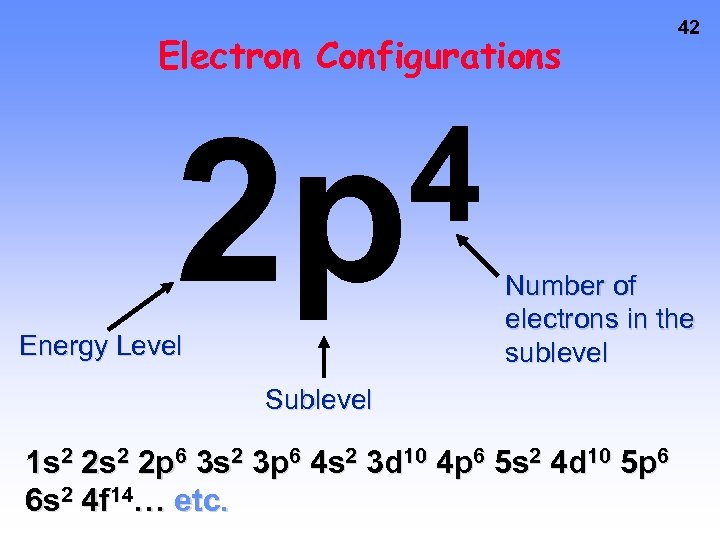Electron Configurations 4 2 p Energy Level 42 Number of electrons in the sublevel Sublevel 1 s 2 2 p 6 3 s 2 3 p 6 4 s 2 3 d 10 4 p 6 5 s 2 4 d 10 5 p 6 6 s 2 4 f 14… etc.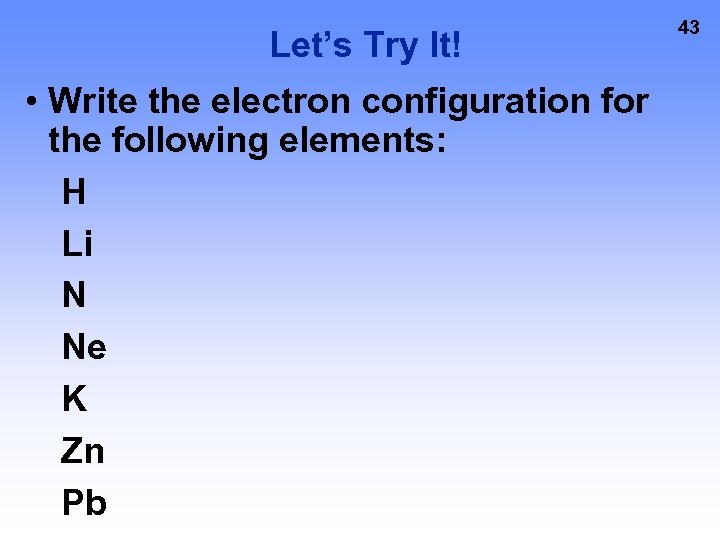Let’s Try It! • Write the electron configuration for the following elements: H Li N Ne K Zn Pb 43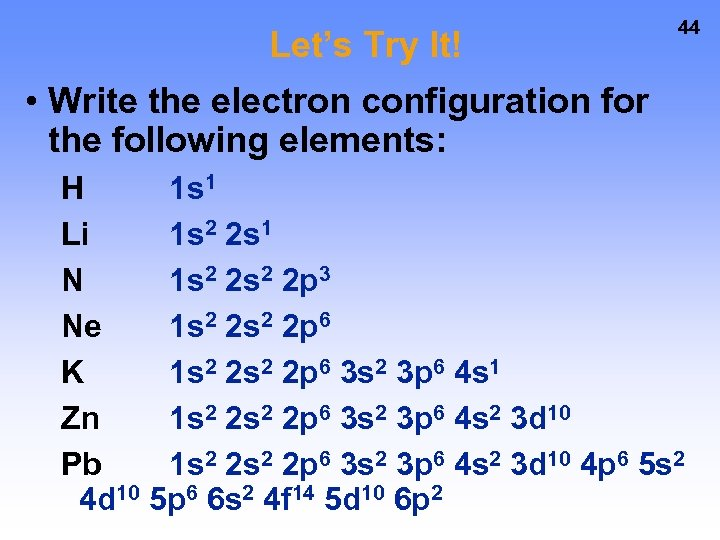Let’s Try It! 44 • Write the electron configuration for the following elements: H 1 s 1 Li 1 s 2 2 s 1 N 1 s 2 2 p 3 Ne 1 s 2 2 p 6 K 1 s 2 2 p 6 3 s 2 3 p 6 4 s 1 Zn 1 s 2 2 p 6 3 s 2 3 p 6 4 s 2 3 d 10 Pb 1 s 2 2 p 6 3 s 2 3 p 6 4 s 2 3 d 10 4 p 6 5 s 2 4 d 10 5 p 6 6 s 2 4 f 14 5 d 10 6 p 2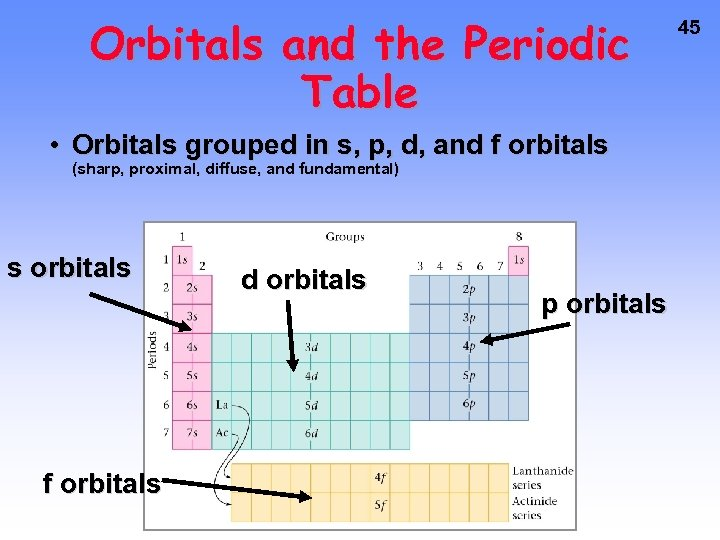Orbitals and the Periodic Table • Orbitals grouped in s, p, d, and f orbitals (sharp, proximal, diffuse, and fundamental) s orbitals f orbitals d orbitals p orbitals 45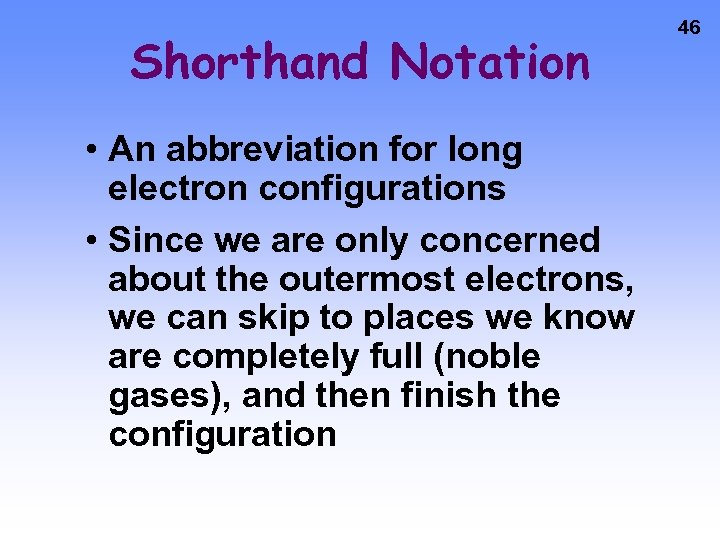Shorthand Notation • An abbreviation for long electron configurations • Since we are only concerned about the outermost electrons, we can skip to places we know are completely full (noble gases), and then finish the configuration 46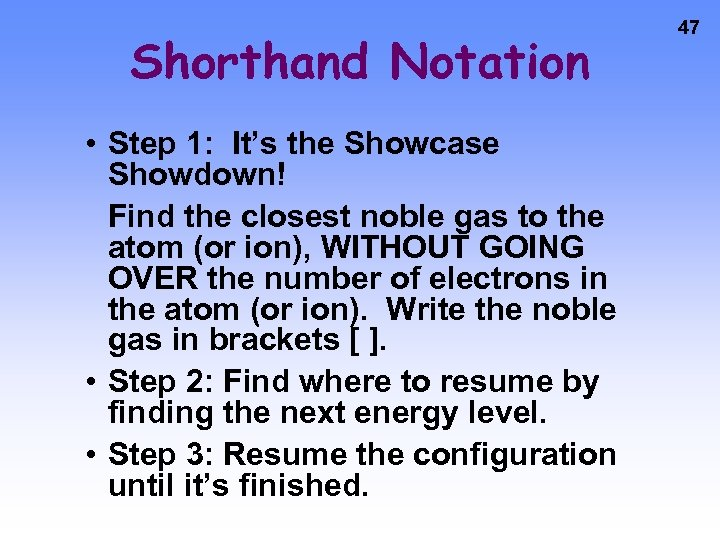Shorthand Notation • Step 1: It’s the Showcase Showdown! Find the closest noble gas to the atom (or ion), WITHOUT GOING OVER the number of electrons in the atom (or ion). Write the noble gas in brackets [ ]. • Step 2: Find where to resume by finding the next energy level. • Step 3: Resume the configuration until it’s finished. 47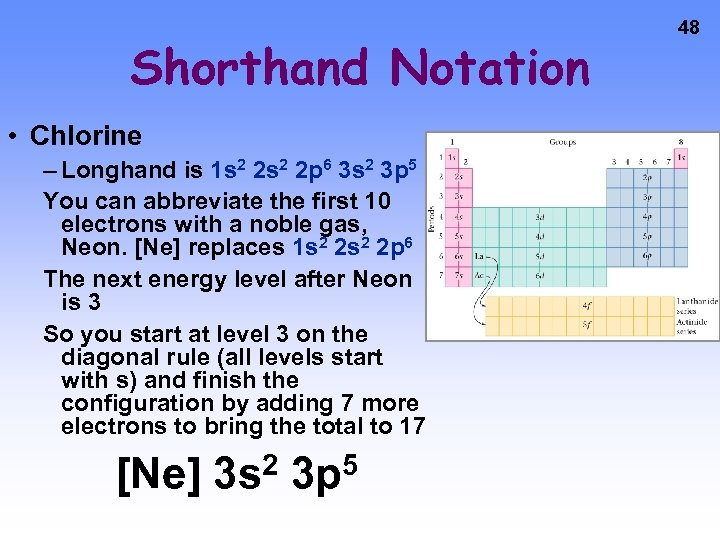Shorthand Notation • Chlorine – Longhand is 1 s 2 2 p 6 3 s 2 3 p 5 You can abbreviate the first 10 electrons with a noble gas, Neon. [Ne] replaces 1 s 2 2 p 6 The next energy level after Neon is 3 So you start at level 3 on the diagonal rule (all levels start with s) and finish the configuration by adding 7 more electrons to bring the total to 17 [Ne] 2 3 s 5 3 p 48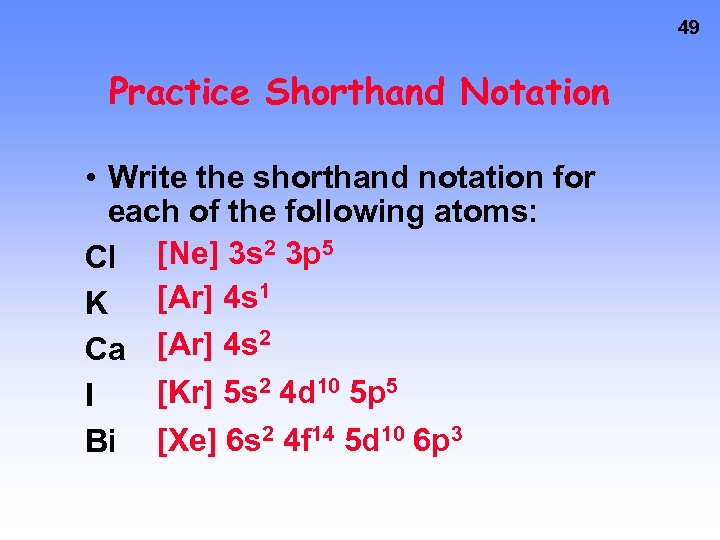49 Practice Shorthand Notation • Write the shorthand notation for each of the following atoms: [Ne] 3 s 2 3 p 5 Cl [Ar] 4 s 1 K [Ar] 4 s 2 Ca [Kr] 5 s 2 4 d 10 5 p 5 I Bi [Xe] 6 s 2 4 f 14 5 d 10 6 p 3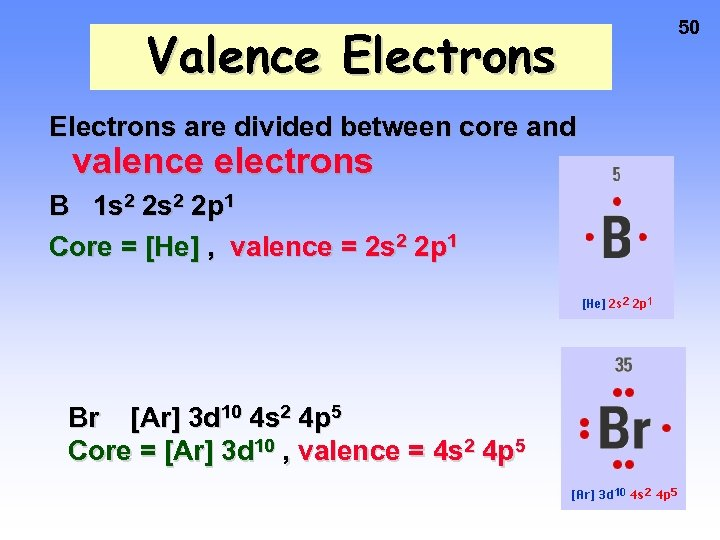Valence Electrons are divided between core and valence electrons B 1 s 2 2 p 1 Core = [He] , valence = 2 s 2 2 p 1 Br [Ar] 3 d 10 4 s 2 4 p 5 Core = [Ar] 3 d 10 , valence = 4 s 2 4 p 5 50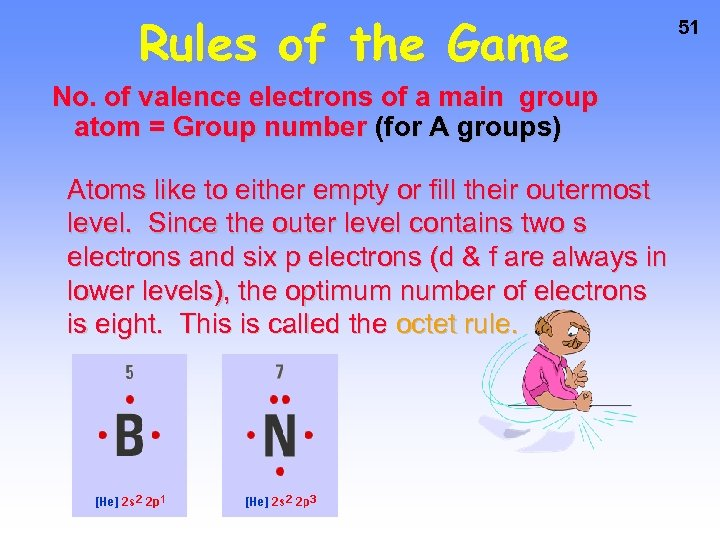Rules of the Game No. of valence electrons of a main group atom = Group number (for A groups) Atoms like to either empty or fill their outermost level. Since the outer level contains two s electrons and six p electrons (d & f are always in lower levels), the optimum number of electrons is eight. This is called the octet rule. 51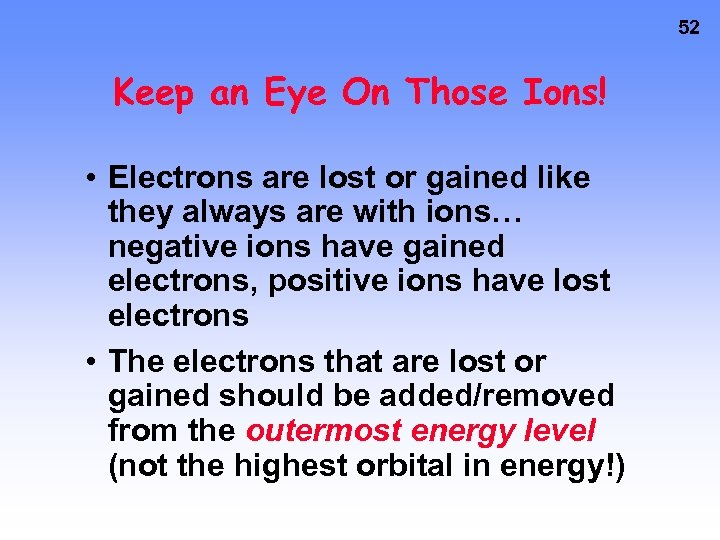52 Keep an Eye On Those Ions! • Electrons are lost or gained like they always are with ions… negative ions have gained electrons, positive ions have lost electrons • The electrons that are lost or gained should be added/removed from the outermost energy level (not the highest orbital in energy!)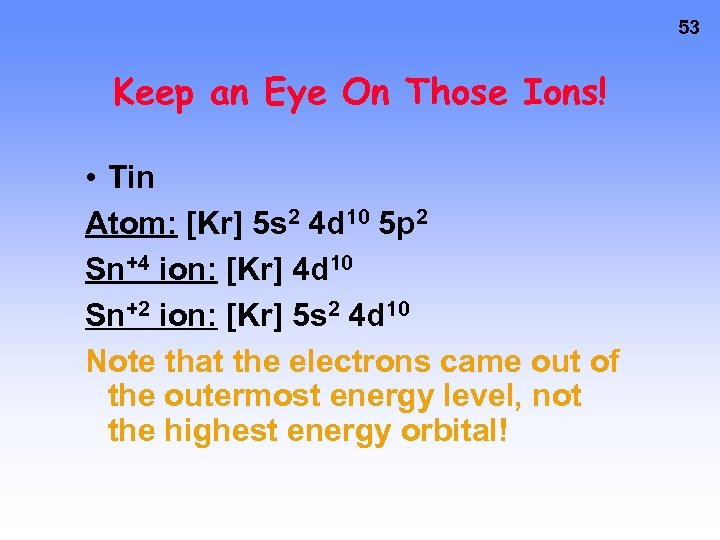53 Keep an Eye On Those Ions! • Tin Atom: [Kr] 5 s 2 4 d 10 5 p 2 Sn+4 ion: [Kr] 4 d 10 Sn+2 ion: [Kr] 5 s 2 4 d 10 Note that the electrons came out of the outermost energy level, not the highest energy orbital!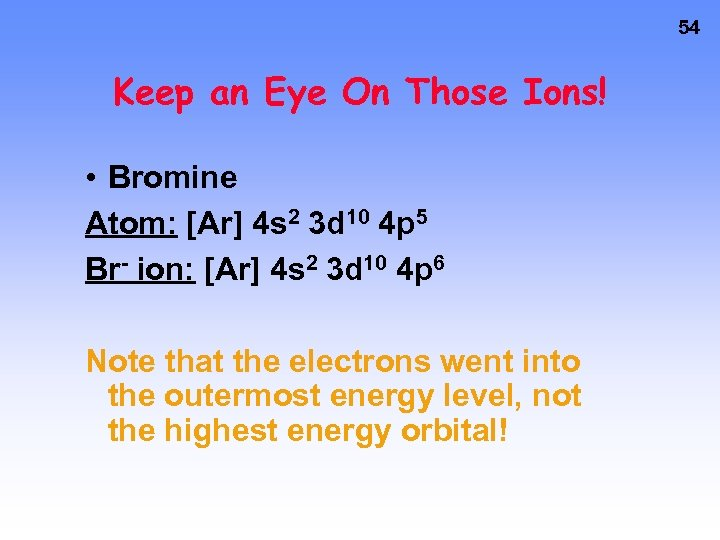54 Keep an Eye On Those Ions! • Bromine Atom: [Ar] 4 s 2 3 d 10 4 p 5 Br- ion: [Ar] 4 s 2 3 d 10 4 p 6 Note that the electrons went into the outermost energy level, not the highest energy orbital!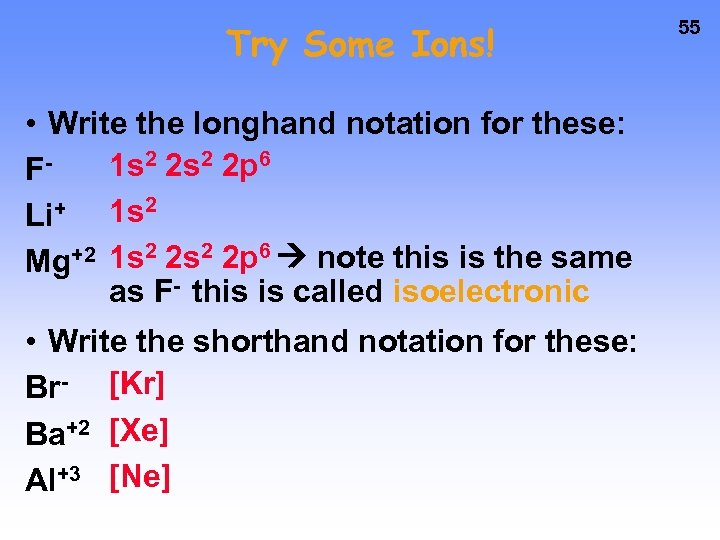Try Some Ions! • Write the longhand notation for these: 1 s 2 2 p 6 FLi+ 1 s 2 Mg+2 1 s 2 2 p 6 note this is the same as F- this is called isoelectronic • Write the shorthand notation for these: Br- [Kr] Ba+2 [Xe] Al+3 [Ne] 55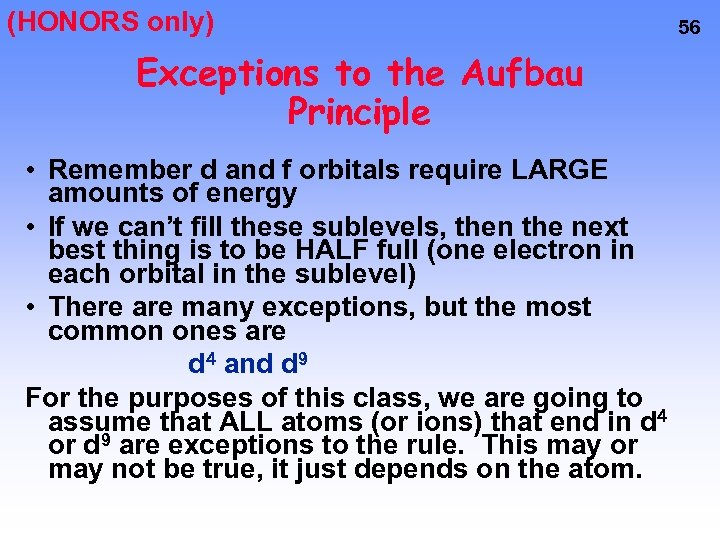(HONORS only) Exceptions to the Aufbau Principle • Remember d and f orbitals require LARGE amounts of energy • If we can’t fill these sublevels, then the next best thing is to be HALF full (one electron in each orbital in the sublevel) • There are many exceptions, but the most common ones are d 4 and d 9 For the purposes of this class, we are going to assume that ALL atoms (or ions) that end in d 4 or d 9 are exceptions to the rule. This may or may not be true, it just depends on the atom. 56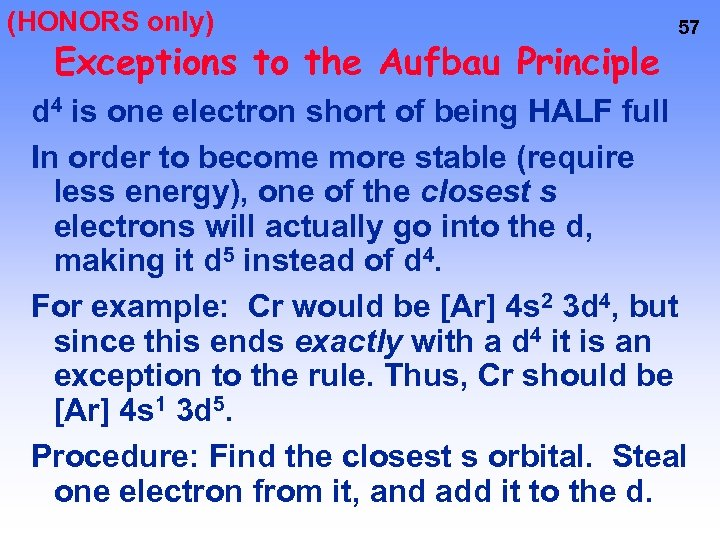(HONORS only) Exceptions to the Aufbau Principle 57 d 4 is one electron short of being HALF full In order to become more stable (require less energy), one of the closest s electrons will actually go into the d, making it d 5 instead of d 4. For example: Cr would be [Ar] 4 s 2 3 d 4, but since this ends exactly with a d 4 it is an exception to the rule. Thus, Cr should be [Ar] 4 s 1 3 d 5. Procedure: Find the closest s orbital. Steal one electron from it, and add it to the d.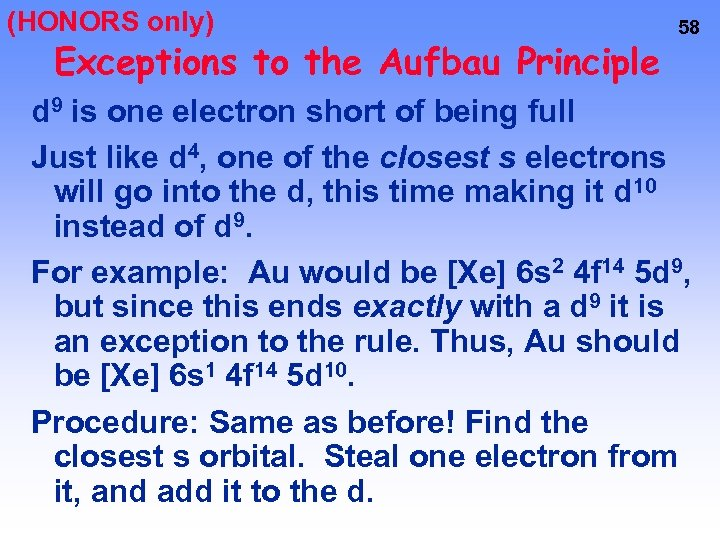(HONORS only) Exceptions to the Aufbau Principle 58 d 9 is one electron short of being full Just like d 4, one of the closest s electrons will go into the d, this time making it d 10 instead of d 9. For example: Au would be [Xe] 6 s 2 4 f 14 5 d 9, but since this ends exactly with a d 9 it is an exception to the rule. Thus, Au should be [Xe] 6 s 1 4 f 14 5 d 10. Procedure: Same as before! Find the closest s orbital. Steal one electron from it, and add it to the d.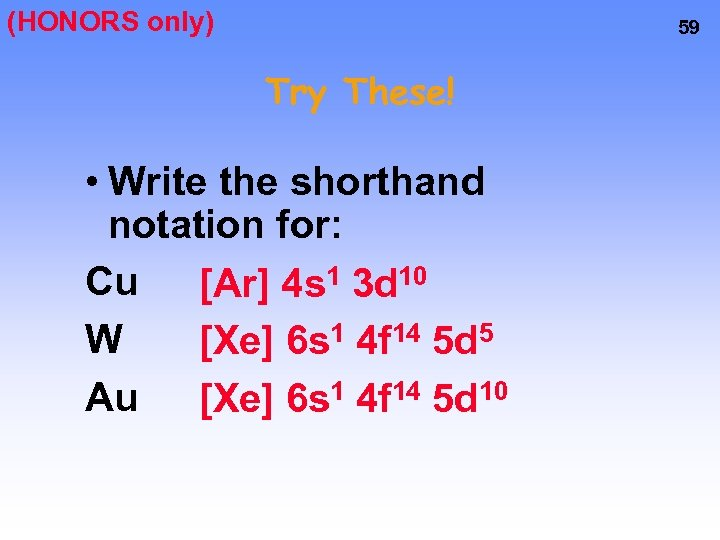(HONORS only) 59 Try These! • Write the shorthand notation for: Cu [Ar] 4 s 1 3 d 10 W [Xe] 6 s 1 4 f 14 5 d 5 Au [Xe] 6 s 1 4 f 14 5 d 10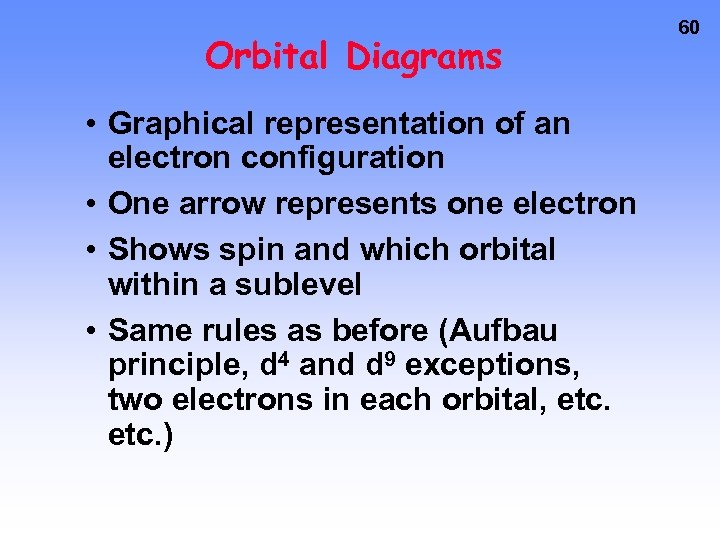Orbital Diagrams • Graphical representation of an electron configuration • One arrow represents one electron • Shows spin and which orbital within a sublevel • Same rules as before (Aufbau principle, d 4 and d 9 exceptions, two electrons in each orbital, etc. ) 60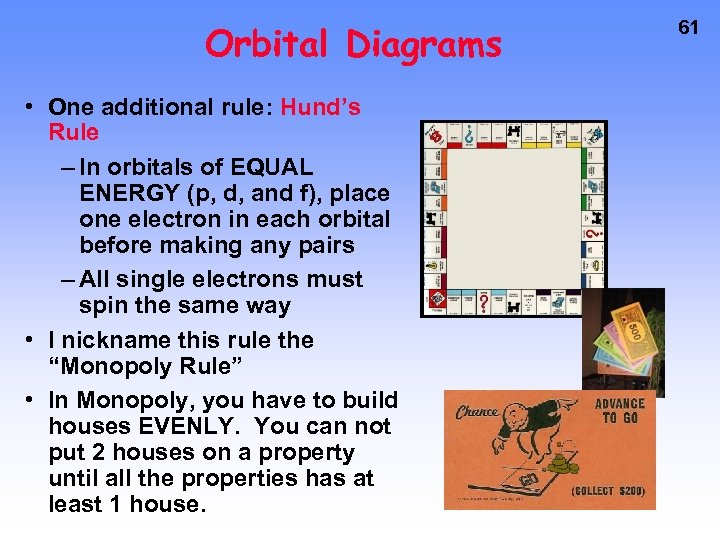Orbital Diagrams • One additional rule: Hund’s Rule – In orbitals of EQUAL ENERGY (p, d, and f), place one electron in each orbital before making any pairs – All single electrons must spin the same way • I nickname this rule the “Monopoly Rule” • In Monopoly, you have to build houses EVENLY. You can not put 2 houses on a property until all the properties has at least 1 house. 61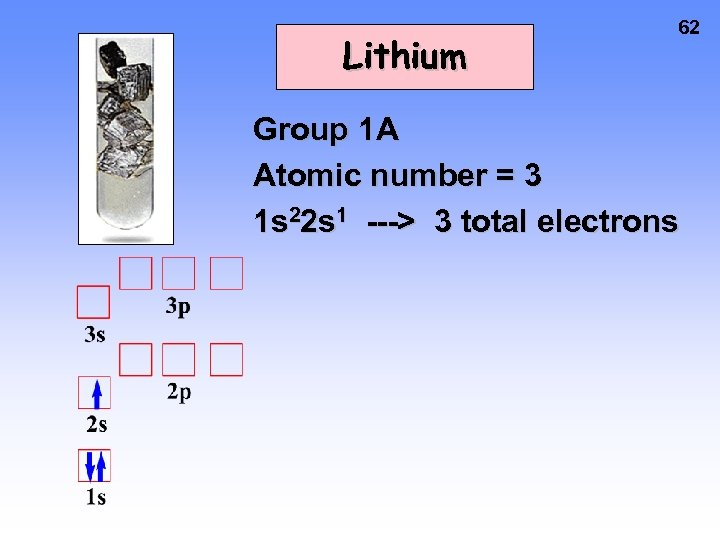Lithium 62 Group 1 A Atomic number = 3 1 s 22 s 1 ---> 3 total electrons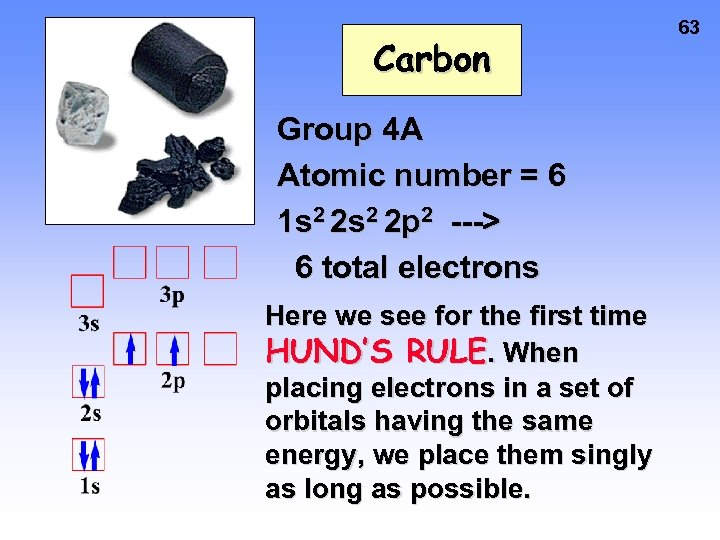Carbon Group 4 A Atomic number = 6 1 s 2 2 p 2 ---> 6 total electrons Here we see for the first time HUND’S RULE. When placing electrons in a set of orbitals having the same energy, we place them singly as long as possible. 63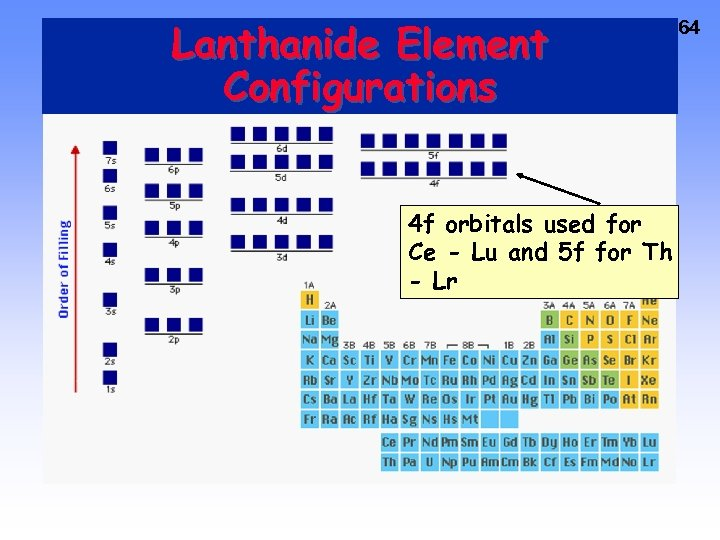Lanthanide Element Configurations 4 f orbitals used for Ce - Lu and 5 f for Th - Lr 64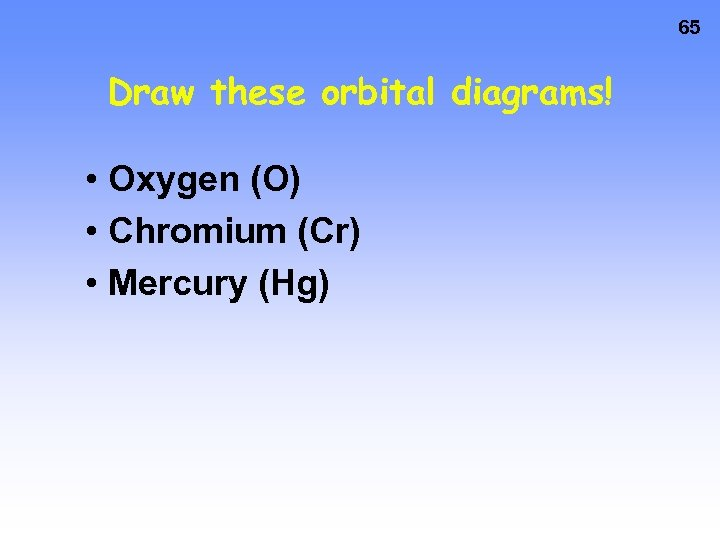65 Draw these orbital diagrams! • Oxygen (O) • Chromium (Cr) • Mercury (Hg)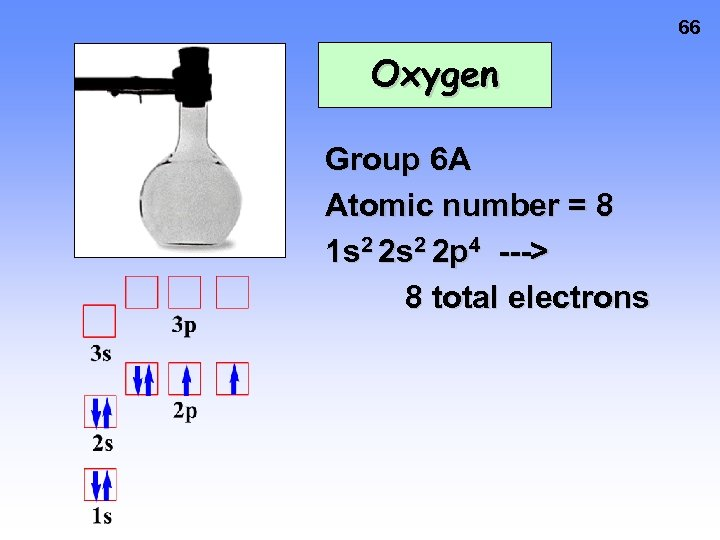66 Oxygen Group 6 A Atomic number = 8 1 s 2 2 p 4 ---> 8 total electrons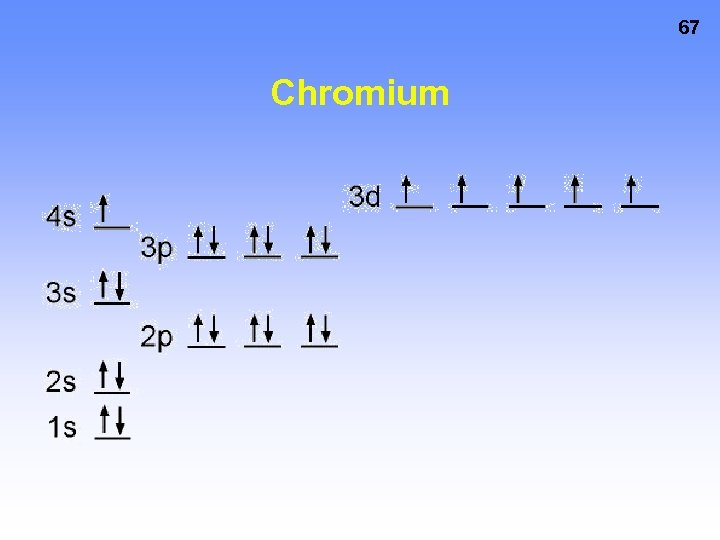67 Chromium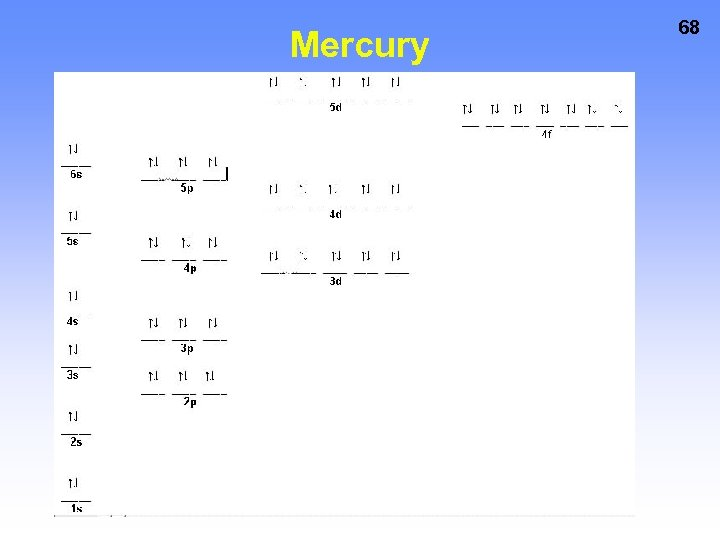Mercury 68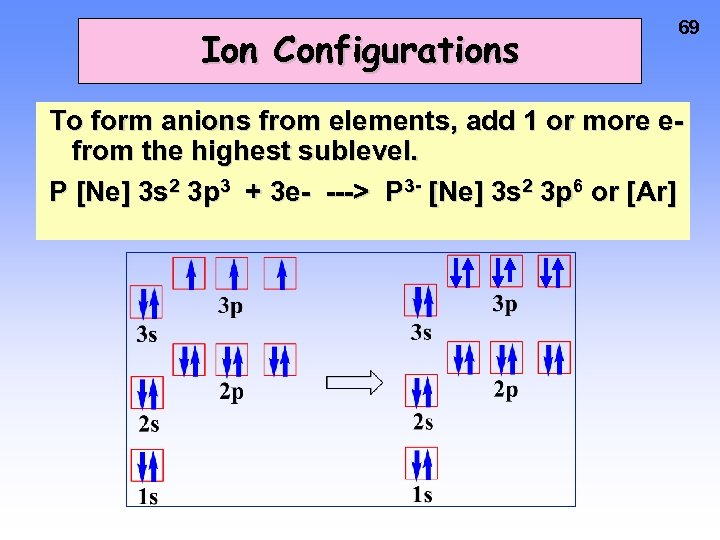Ion Configurations 69 To form anions from elements, add 1 or more efrom the highest sublevel. P [Ne] 3 s 2 3 p 3 + 3 e- ---> P 3 - [Ne] 3 s 2 3 p 6 or [Ar]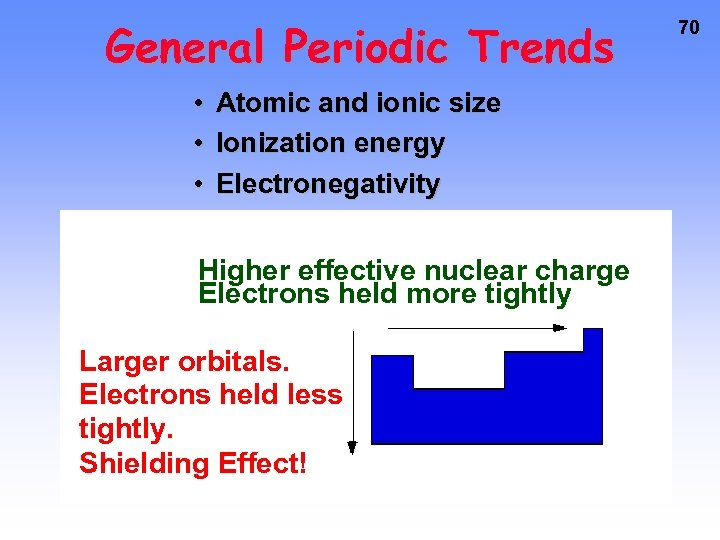General Periodic Trends • Atomic and ionic size • Ionization energy • Electronegativity Higher effective nuclear charge Electrons held more tightly Larger orbitals. Electrons held less tightly. Shielding Effect! 70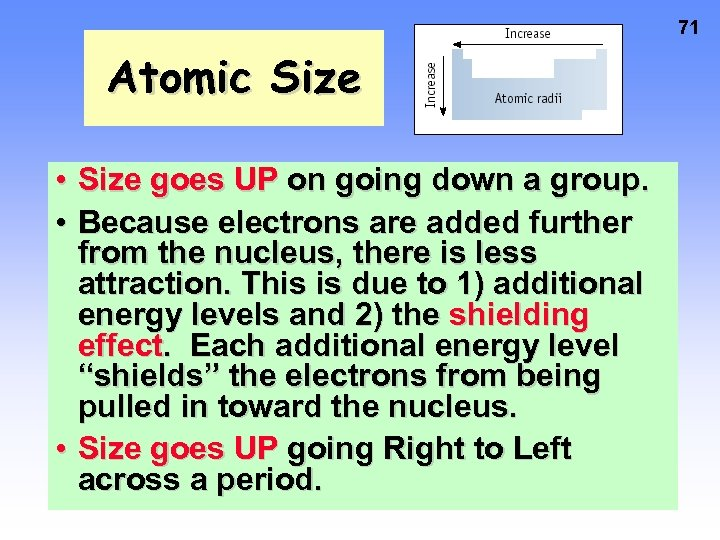71 Atomic Size • Size goes UP on going down a group. • Because electrons are added further from the nucleus, there is less attraction. This is due to 1) additional energy levels and 2) the shielding effect. Each additional energy level “shields” the electrons from being pulled in toward the nucleus. • Size goes UP going Right to Left across a period.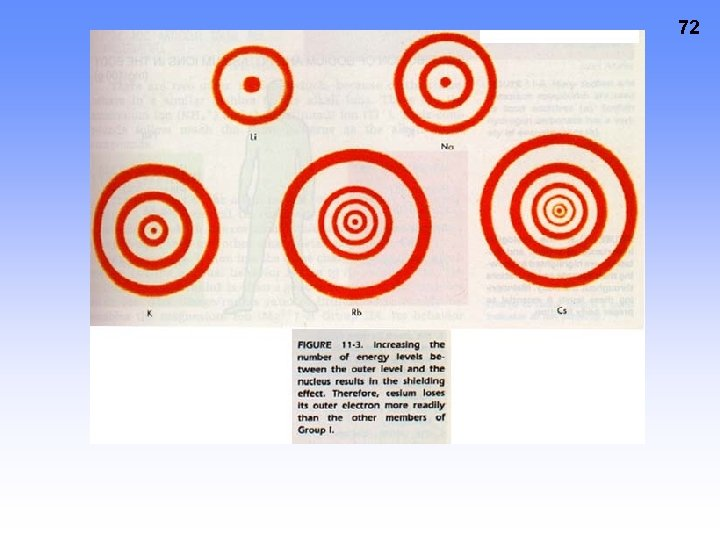72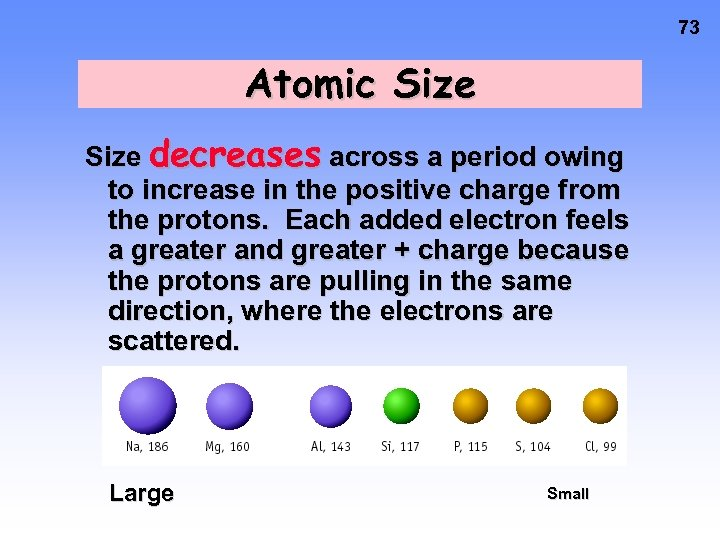73 Atomic Size decreases across a period owing to increase in the positive charge from the protons. Each added electron feels a greater and greater + charge because the protons are pulling in the same direction, where the electrons are scattered. Large Small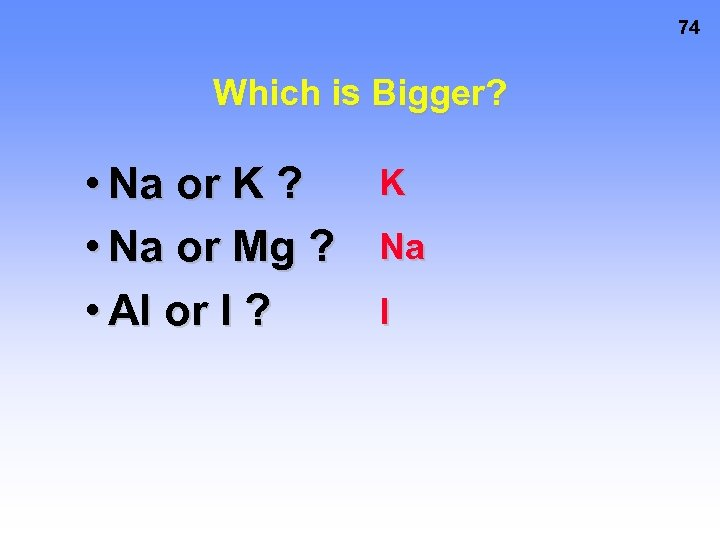74 Which is Bigger? • Na or K ? • Na or Mg ? • Al or I ? K Na I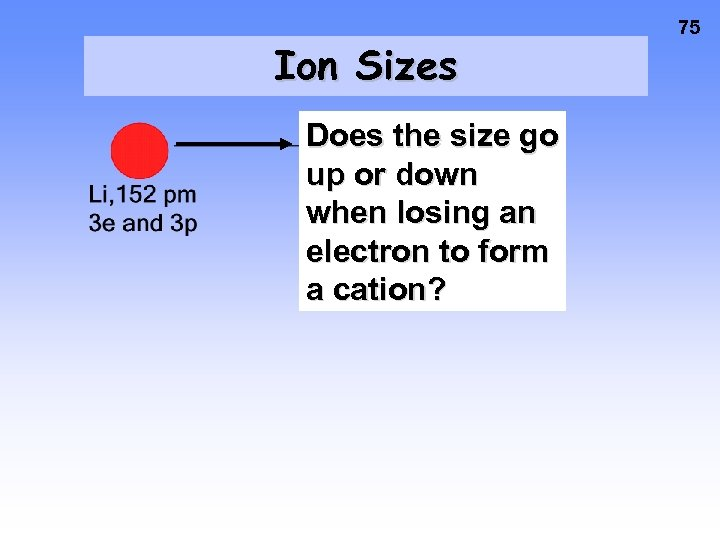Ion Sizes Does the size go up or down when losing an electron to form a cation? 75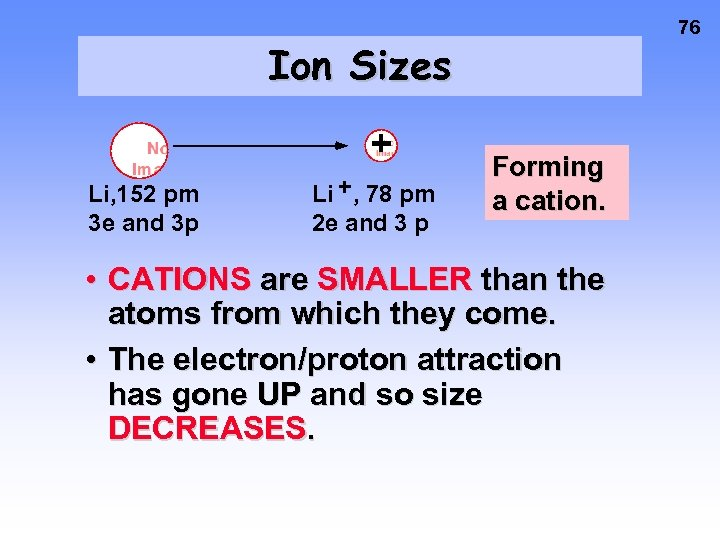76 Ion Sizes + Li, 152 pm 3 e and 3 p Li + , 78 pm 2 e and 3 p Forming a cation. • CATIONS are SMALLER than the atoms from which they come. • The electron/proton attraction has gone UP and so size DECREASES.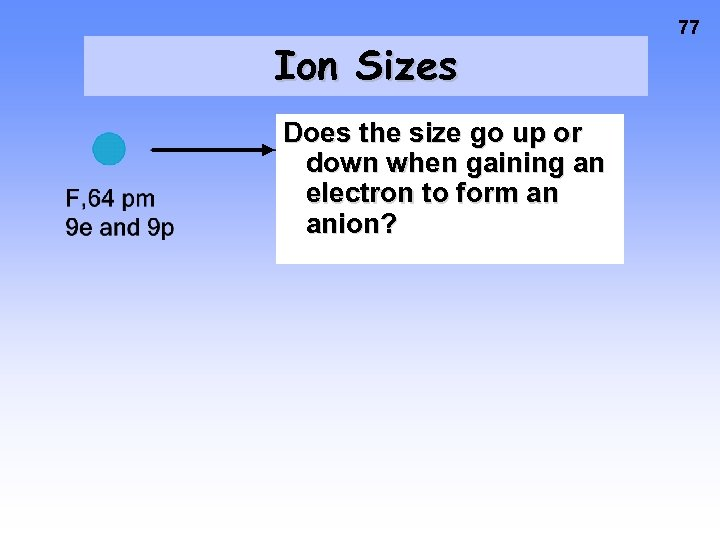Ion Sizes Does the size go up or down when gaining an electron to form an anion? 77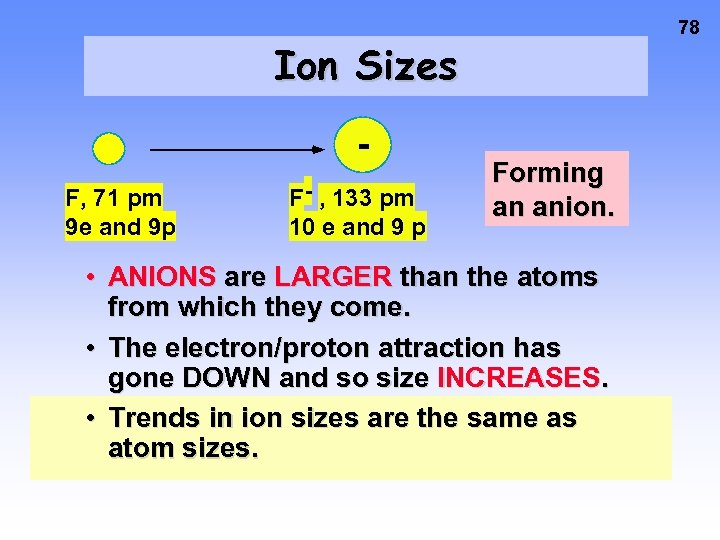78 Ion Sizes F, 71 pm 9 e and 9 p F- , 133 pm 10 e and 9 p Forming an anion. • ANIONS are LARGER than the atoms from which they come. • The electron/proton attraction has gone DOWN and so size INCREASES. • Trends in ion sizes are the same as atom sizes.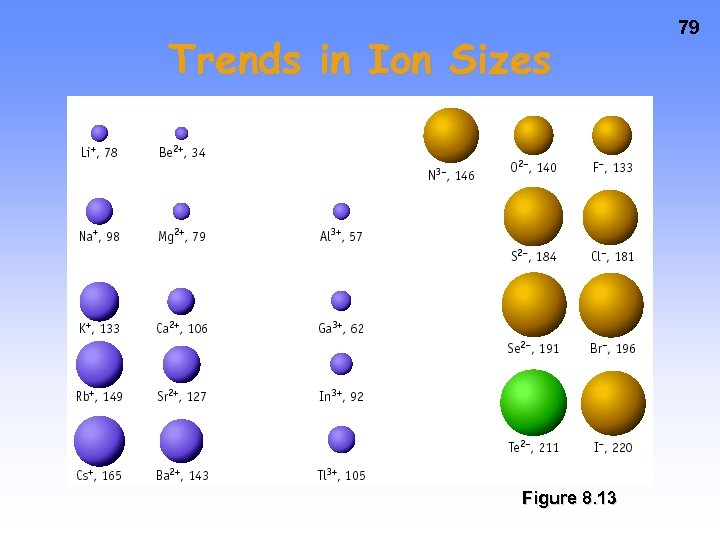Trends in Ion Sizes Figure 8. 13 79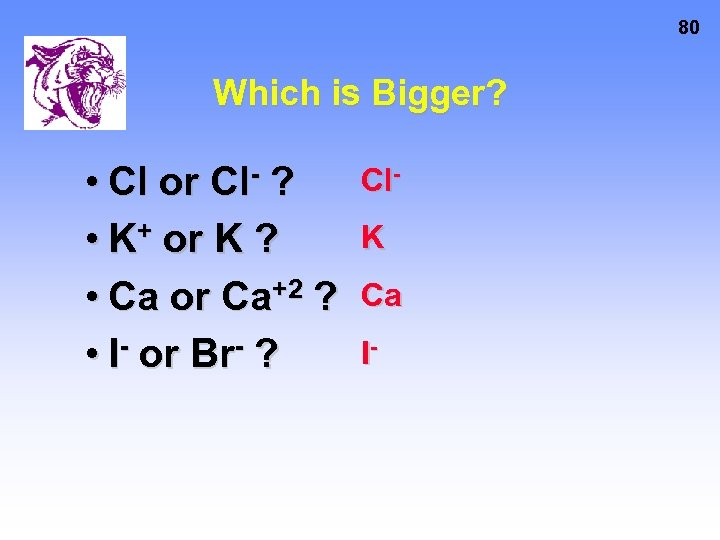80 Which is Bigger? • Cl or Cl- ? • K+ or K ? • Ca or Ca+2 ? • I- or Br- ? Cl. K Ca I-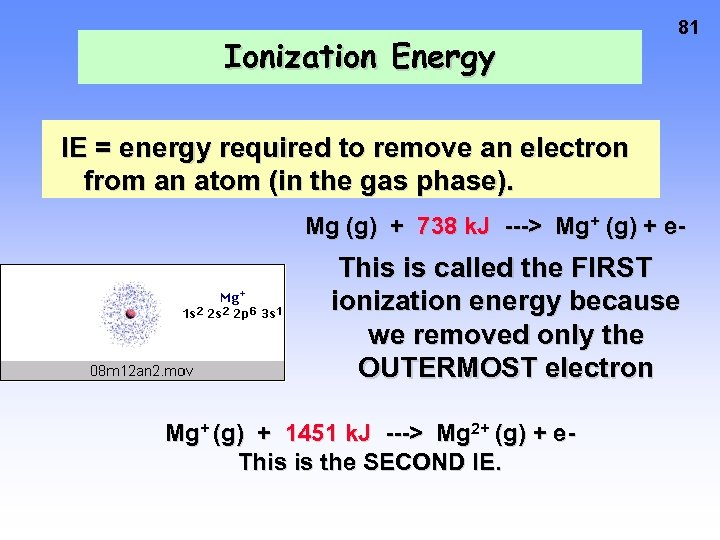Ionization Energy 81 IE = energy required to remove an electron from an atom (in the gas phase). Mg (g) + 738 k. J ---> Mg+ (g) + e- This is called the FIRST ionization energy because we removed only the OUTERMOST electron Mg+ (g) + 1451 k. J ---> Mg 2+ (g) + e. This is the SECOND IE.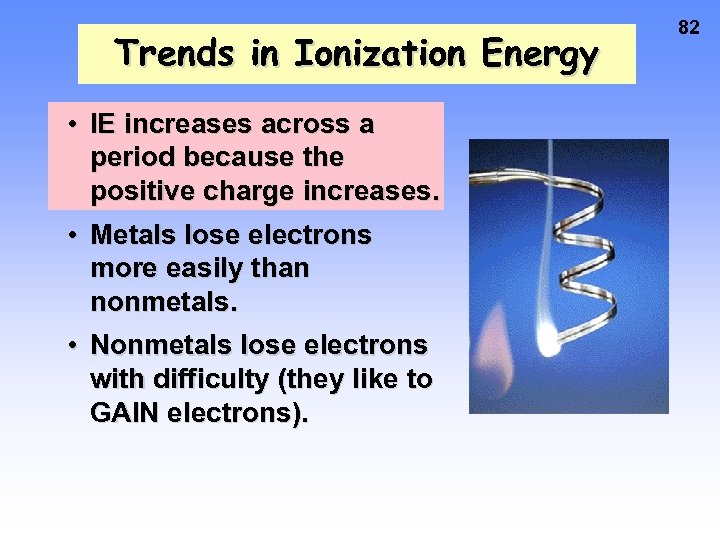Trends in Ionization Energy • IE increases across a period because the positive charge increases. • Metals lose electrons more easily than nonmetals. • Nonmetals lose electrons with difficulty (they like to GAIN electrons). 82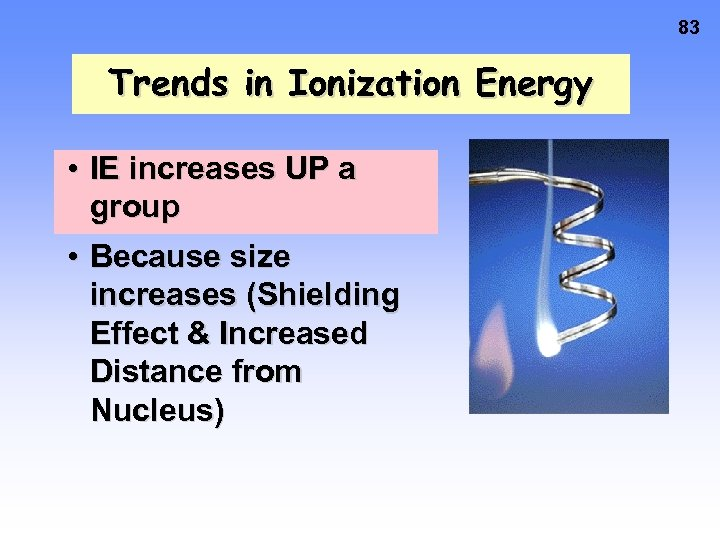83 Trends in Ionization Energy • IE increases UP a group • Because size increases (Shielding Effect & Increased Distance from Nucleus)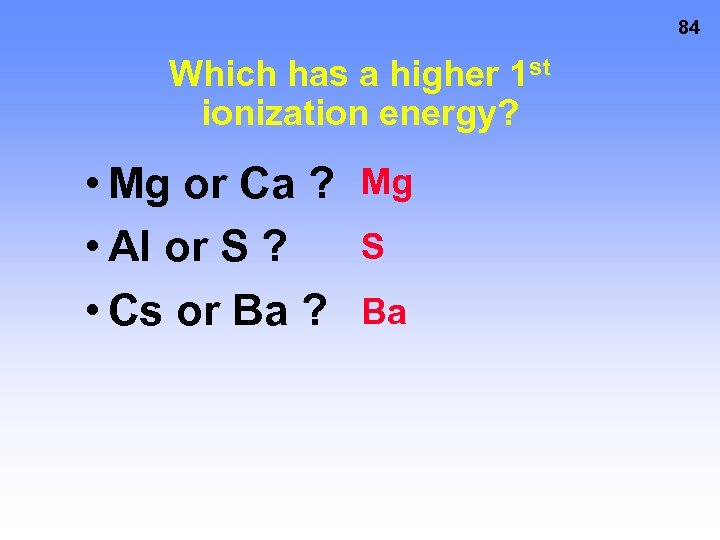84 Which has a higher 1 st ionization energy? • Mg or Ca ? Mg S • Al or S ? • Cs or Ba ? Ba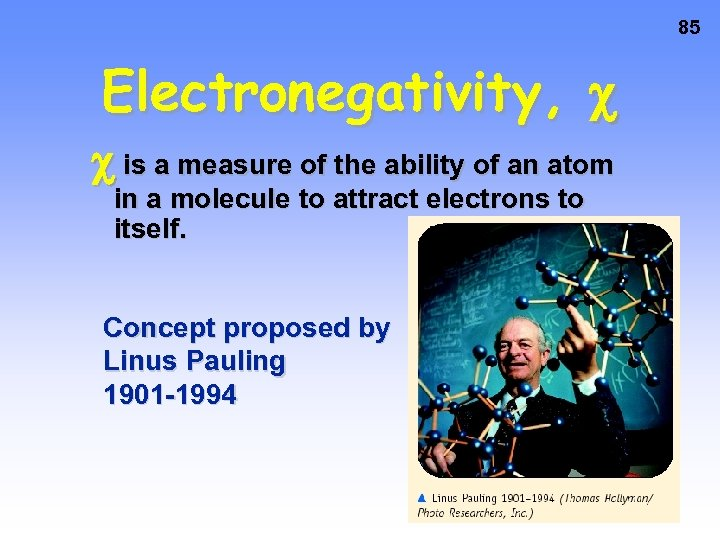85 Electronegativity, is a measure of the ability of an atom in a molecule to attract electrons to itself. Concept proposed by Linus Pauling 1901 -1994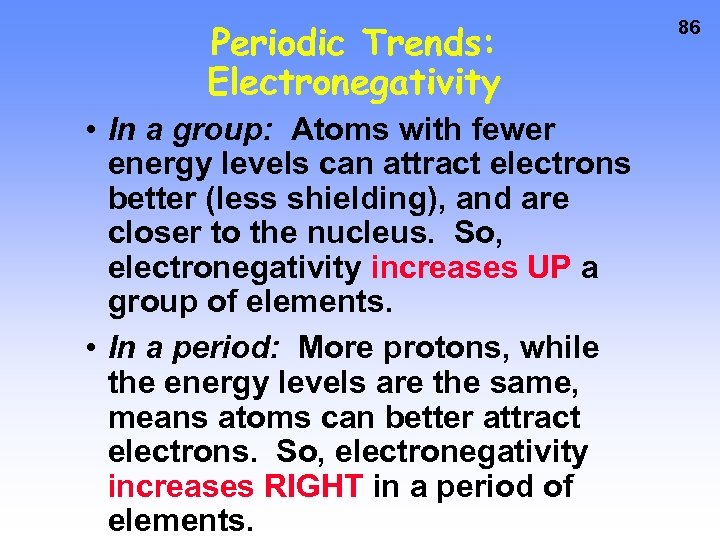Periodic Trends: Electronegativity • In a group: Atoms with fewer energy levels can attract electrons better (less shielding), and are closer to the nucleus. So, electronegativity increases UP a group of elements. • In a period: More protons, while the energy levels are the same, means atoms can better attract electrons. So, electronegativity increases RIGHT in a period of elements. 86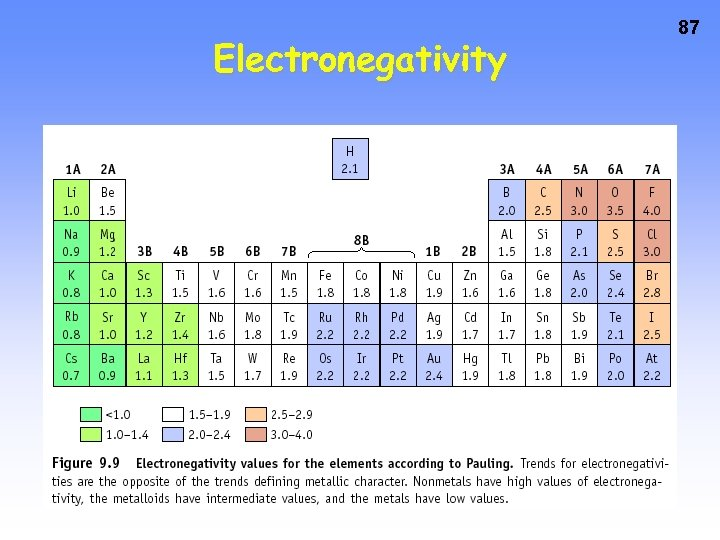Electronegativity 87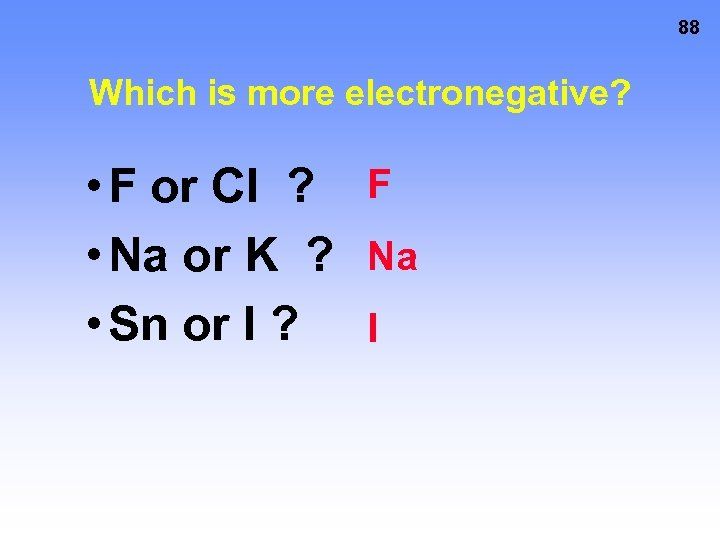88 Which is more electronegative? • F or Cl ? F • Na or K ? Na • Sn or I ? I89 The End !!!!!!!!!!